# RD Sharma Solutions for Class 8 Maths Chapter 17 Understanding Shapes- III (Special Types of Quadrilaterals)

In Chapter 17 of RD Sharma Class 8 Maths, we shall learn concepts based on some special types of quadrilaterals and their properties. The experienced faculty team at BYJU’S has explained the concepts in a step by step format to help students analyze their problem-solving ability when preparing for their board exams. Students are advised to practice on a regular basis which helps them crack even difficult questions at ease in their examinations. RD Sharma Class 8 is one of the best study materials one opts for their exam preparation, which yields good results. Students can download the PDF from the links given below and start practising.

Chapter 17- Understanding Shapes-III (Special Types of Quadrilaterals) contains three exercises and the RD Sharma Solutions present in this page provide solutions to the questions present in each exercise. Now, let us have a look at the concepts discussed in this chapter.

• Various types of quadrilaterals – trapezium, isosceles trapezium, parallelogram, rhombus, rectangle, square.
• Properties of a parallelogram.
• Properties of a rhombus.
• Properties of a rectangle.
• Properties of a square.
• Properties of a trapezium.

## Download the Pdf of RD Sharma Solutions for Class 8 Maths Chapter 17 Understanding Shapes- III (Special Types of Quadrilaterals)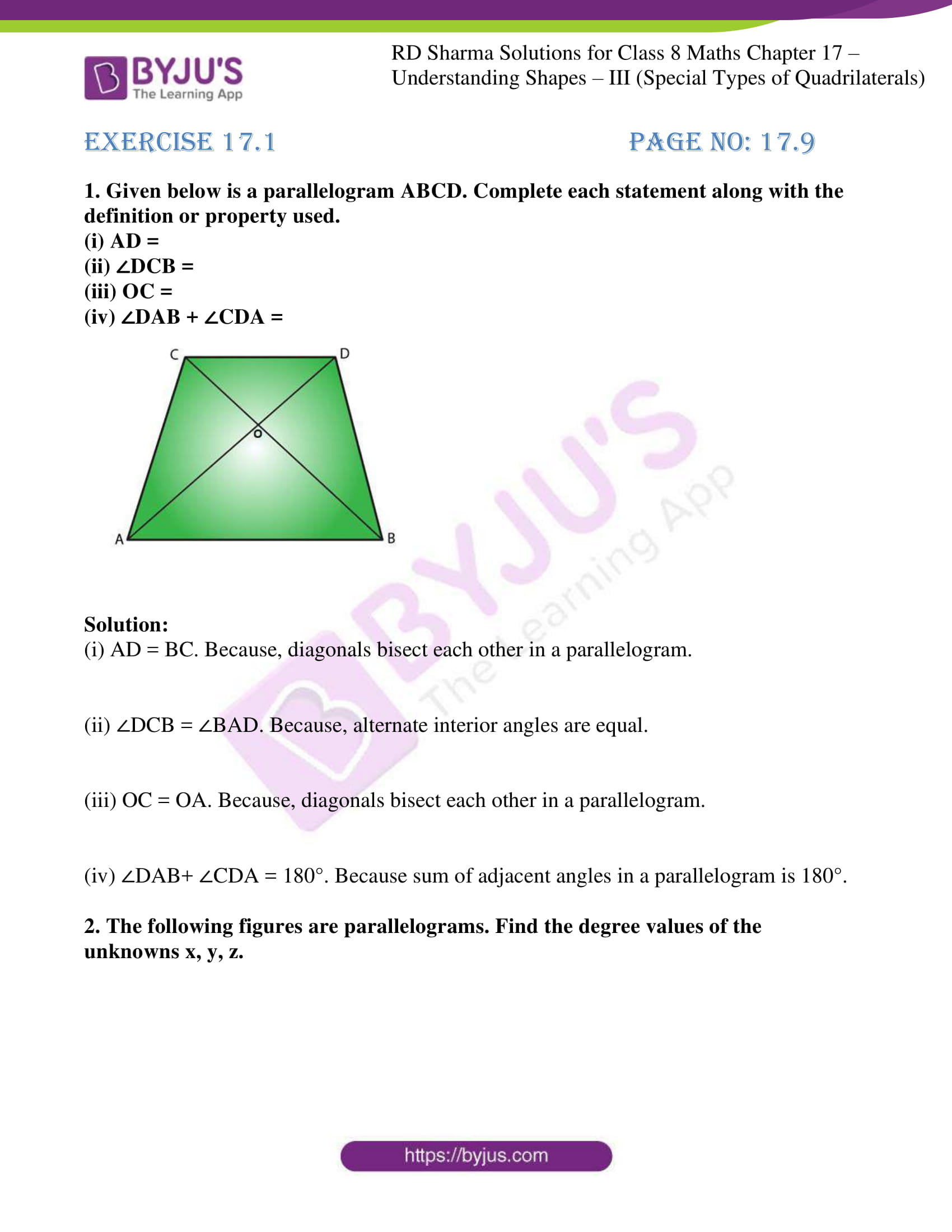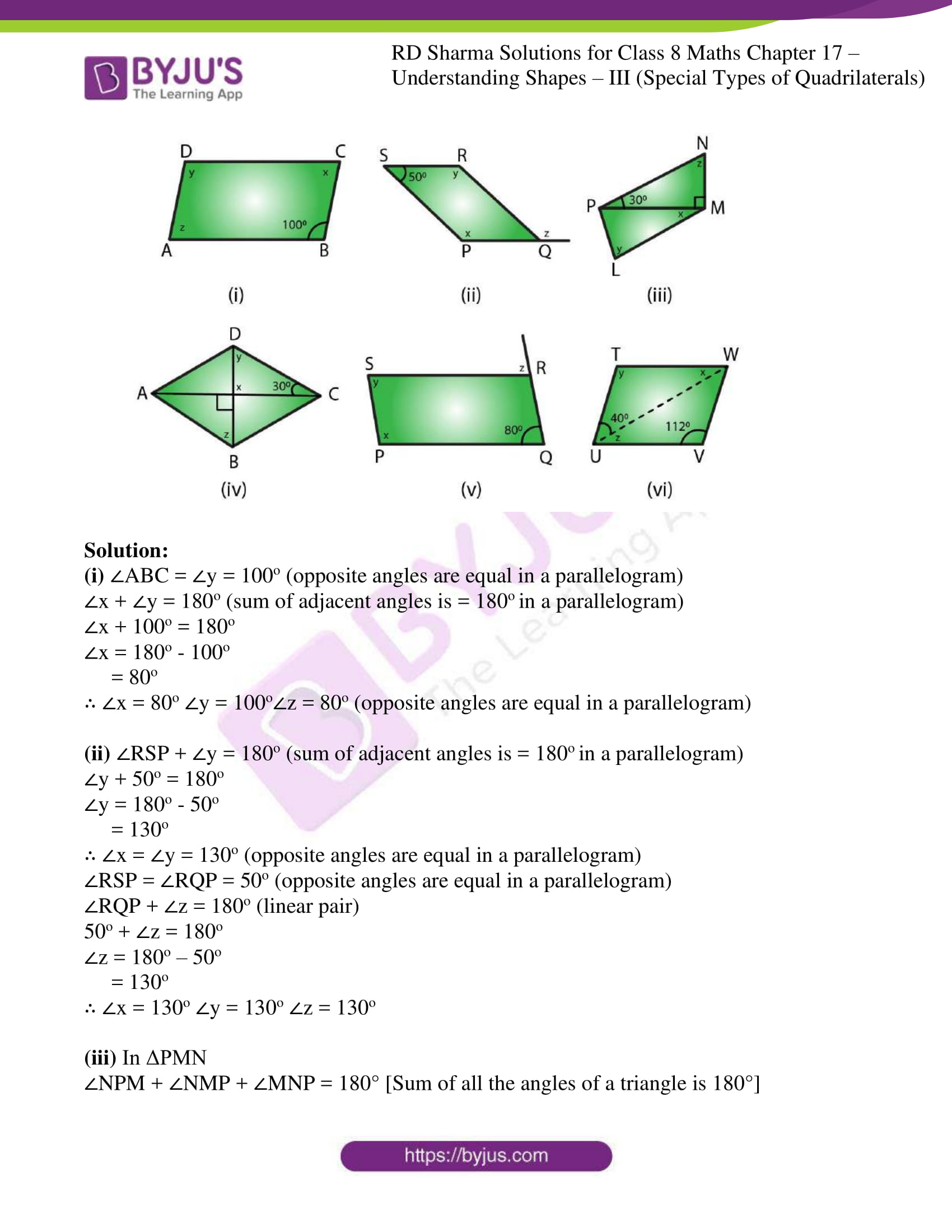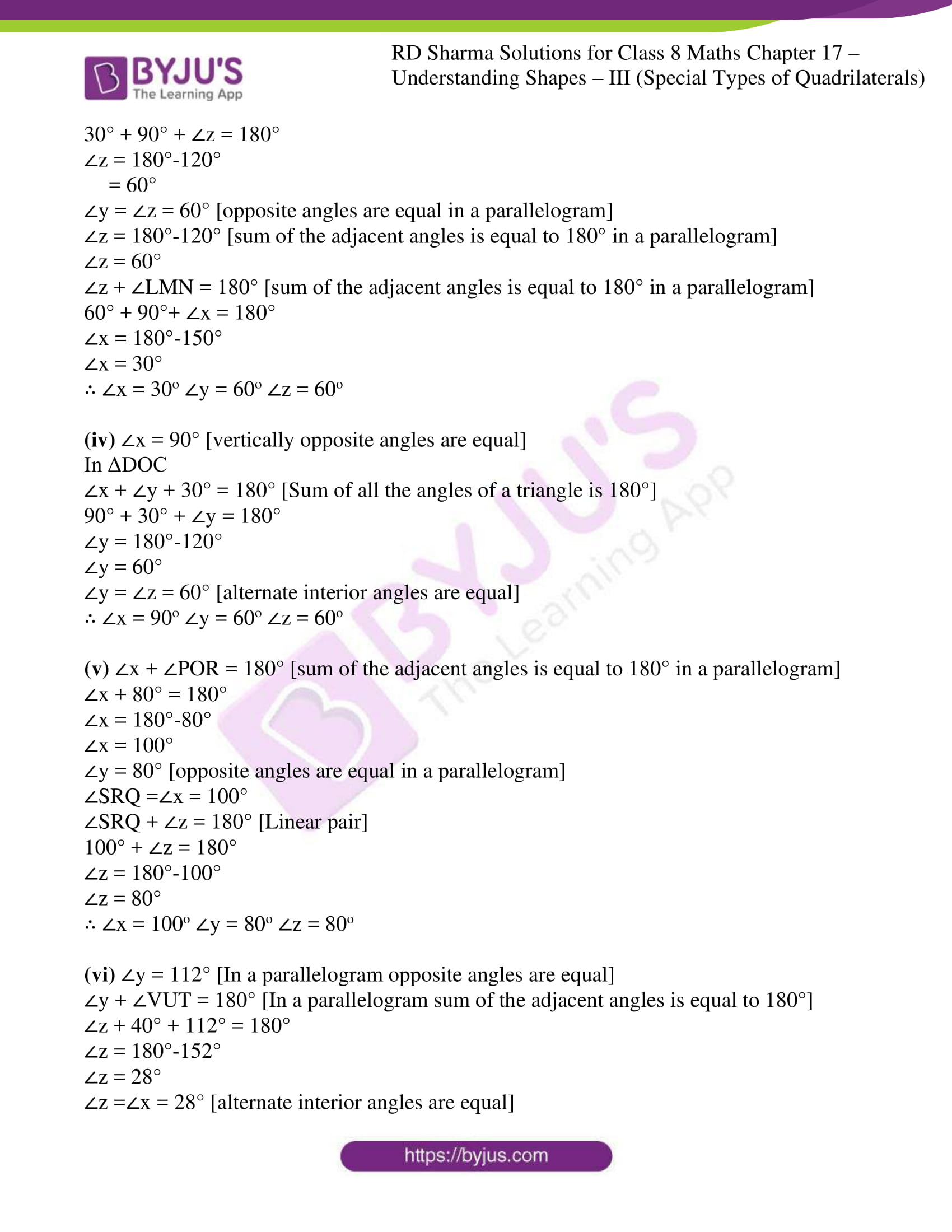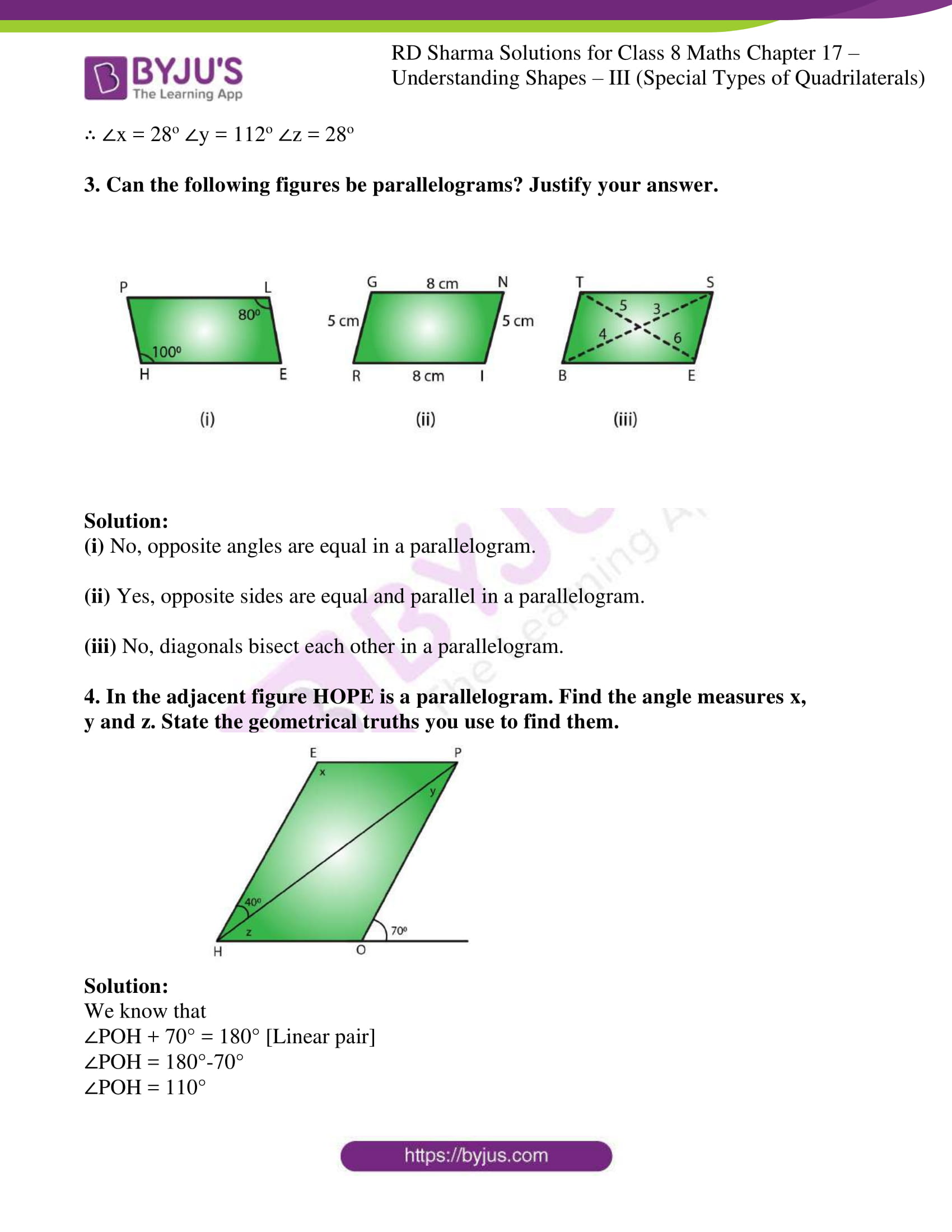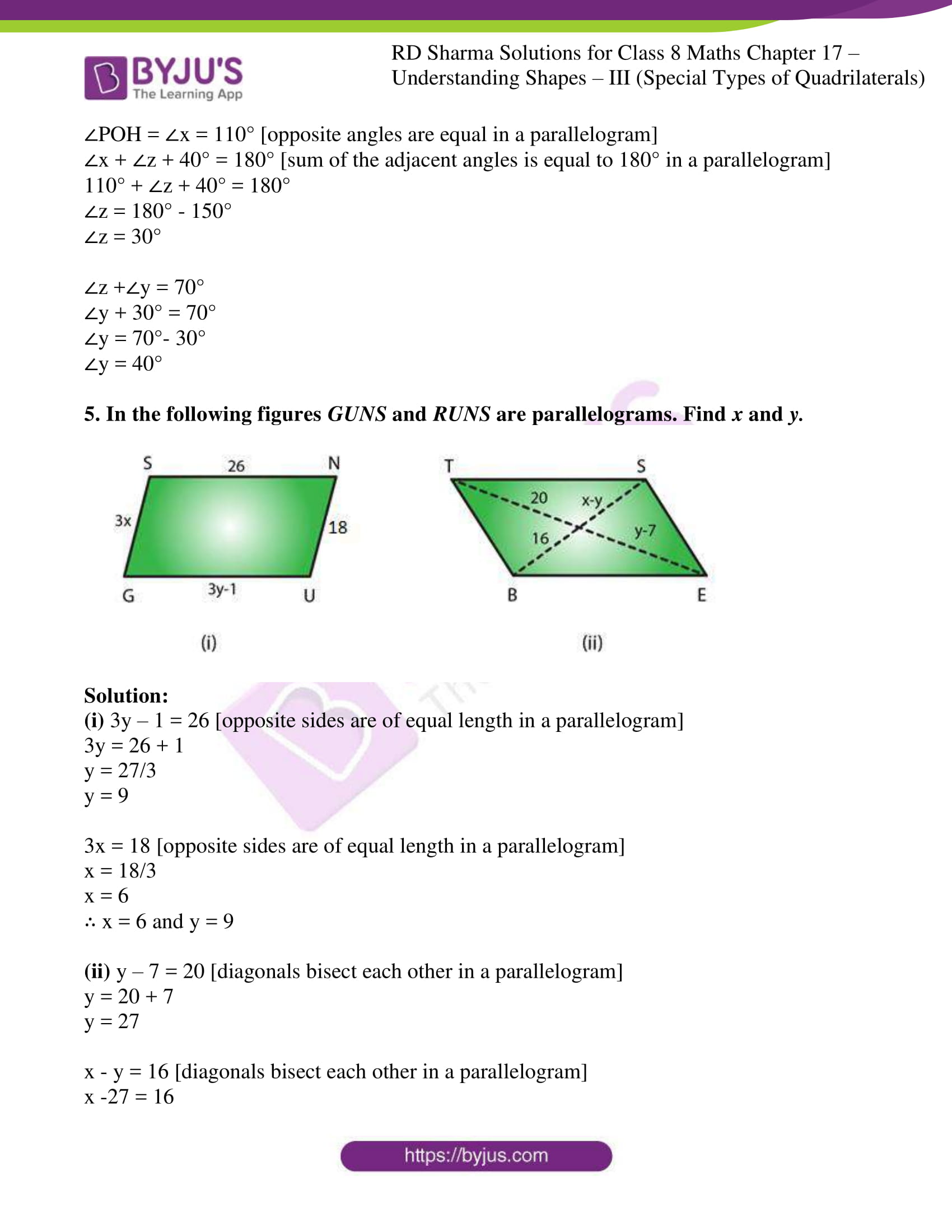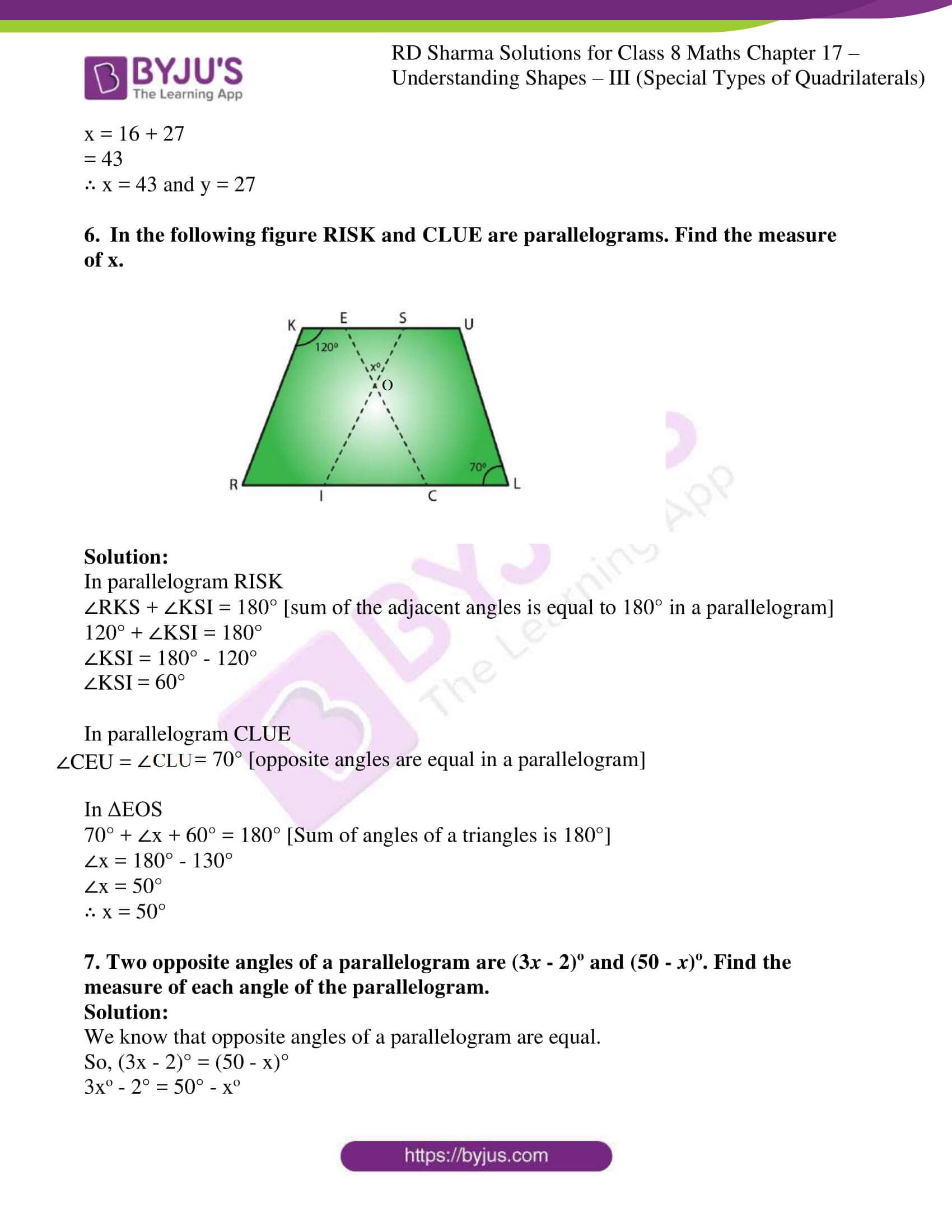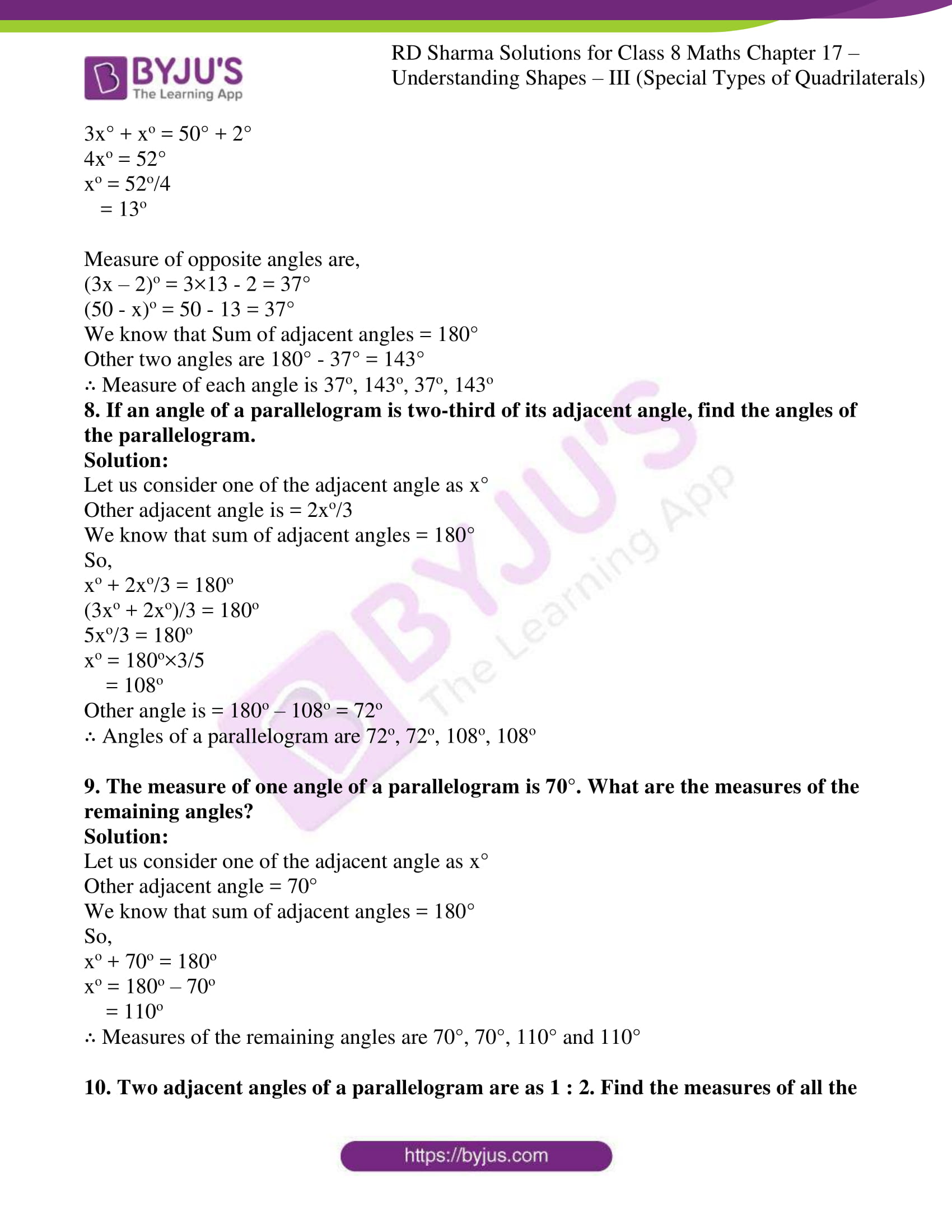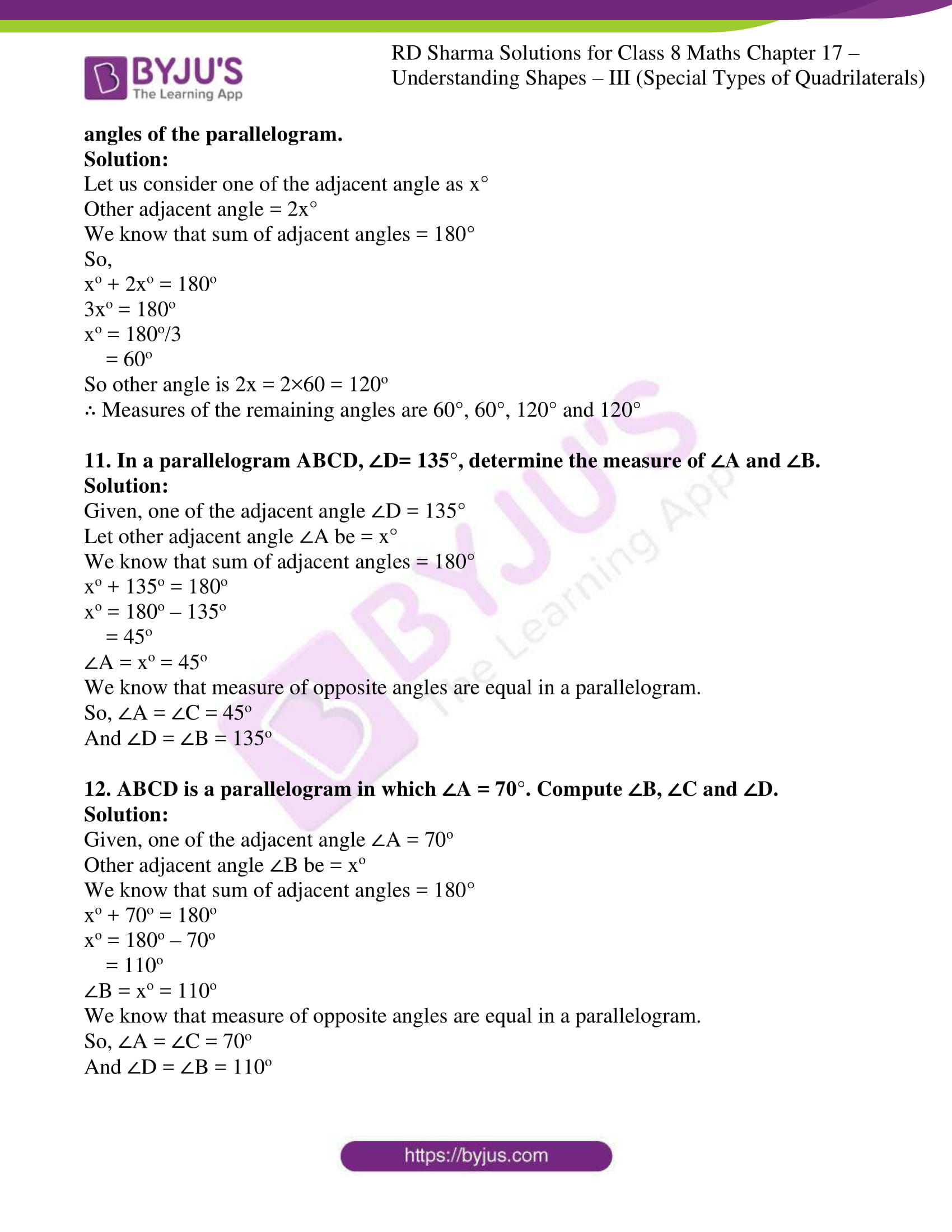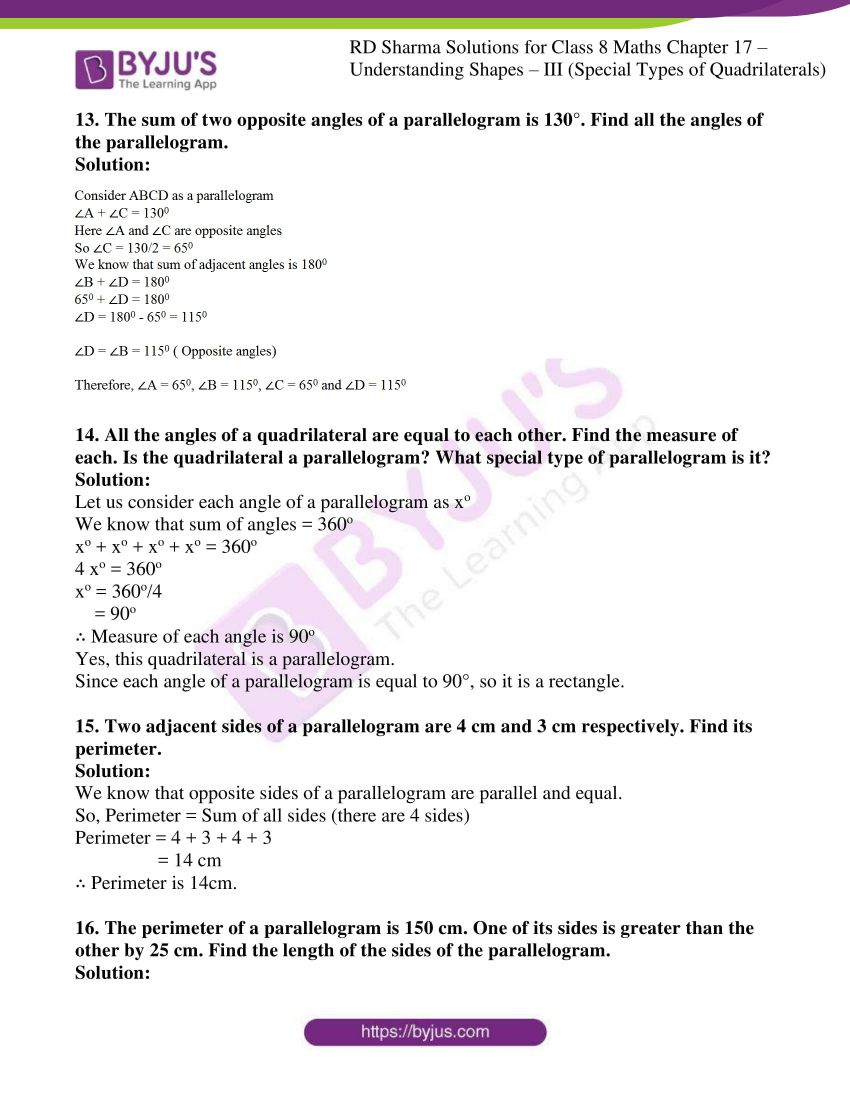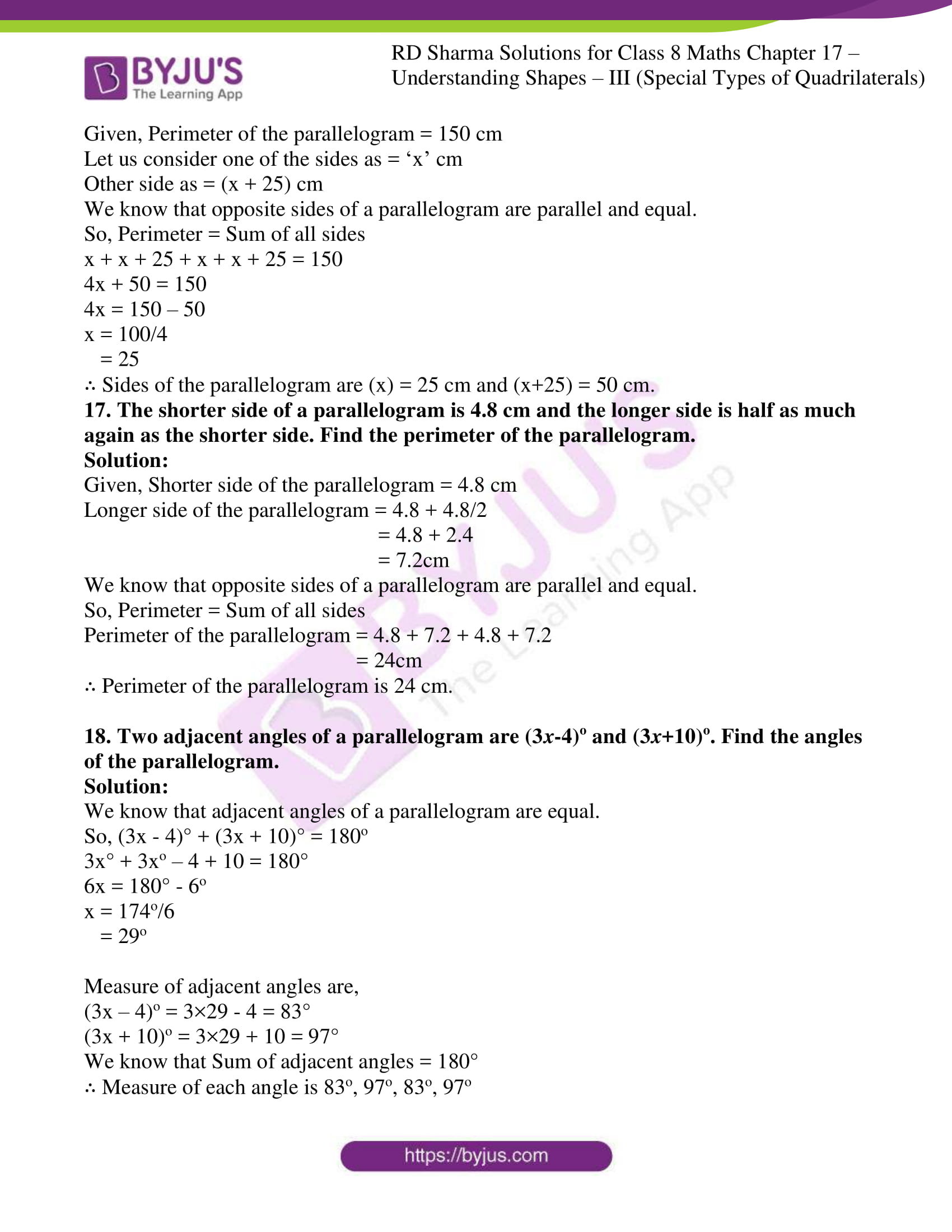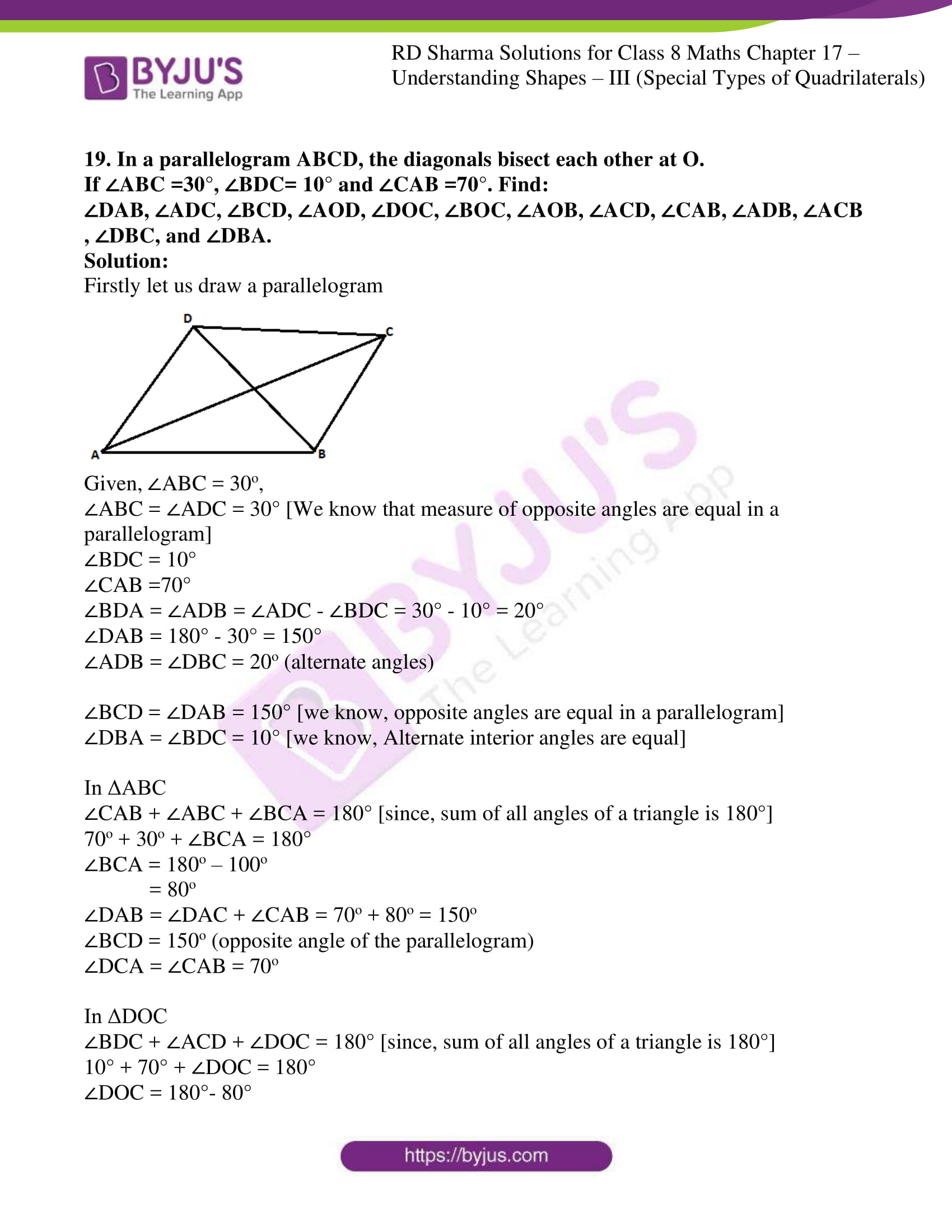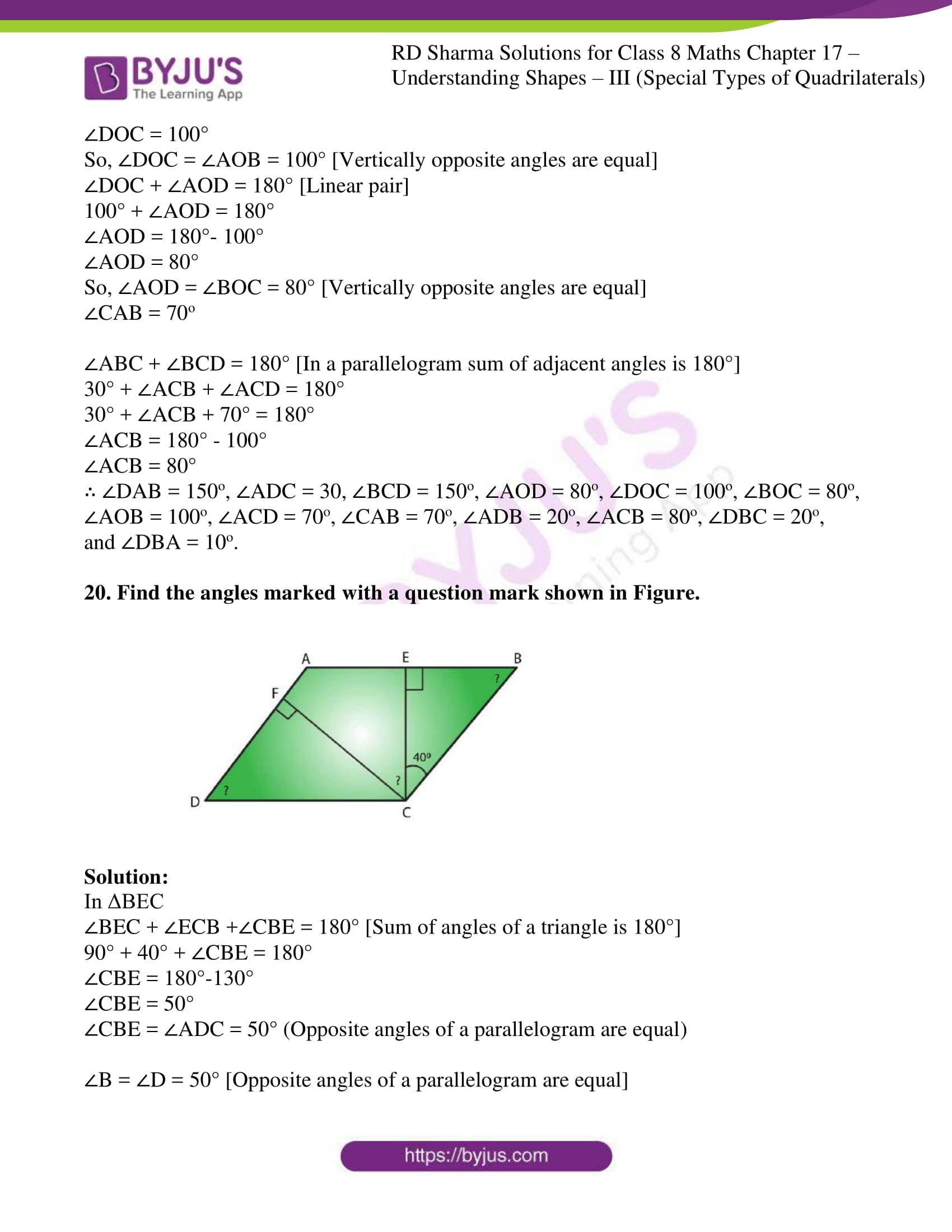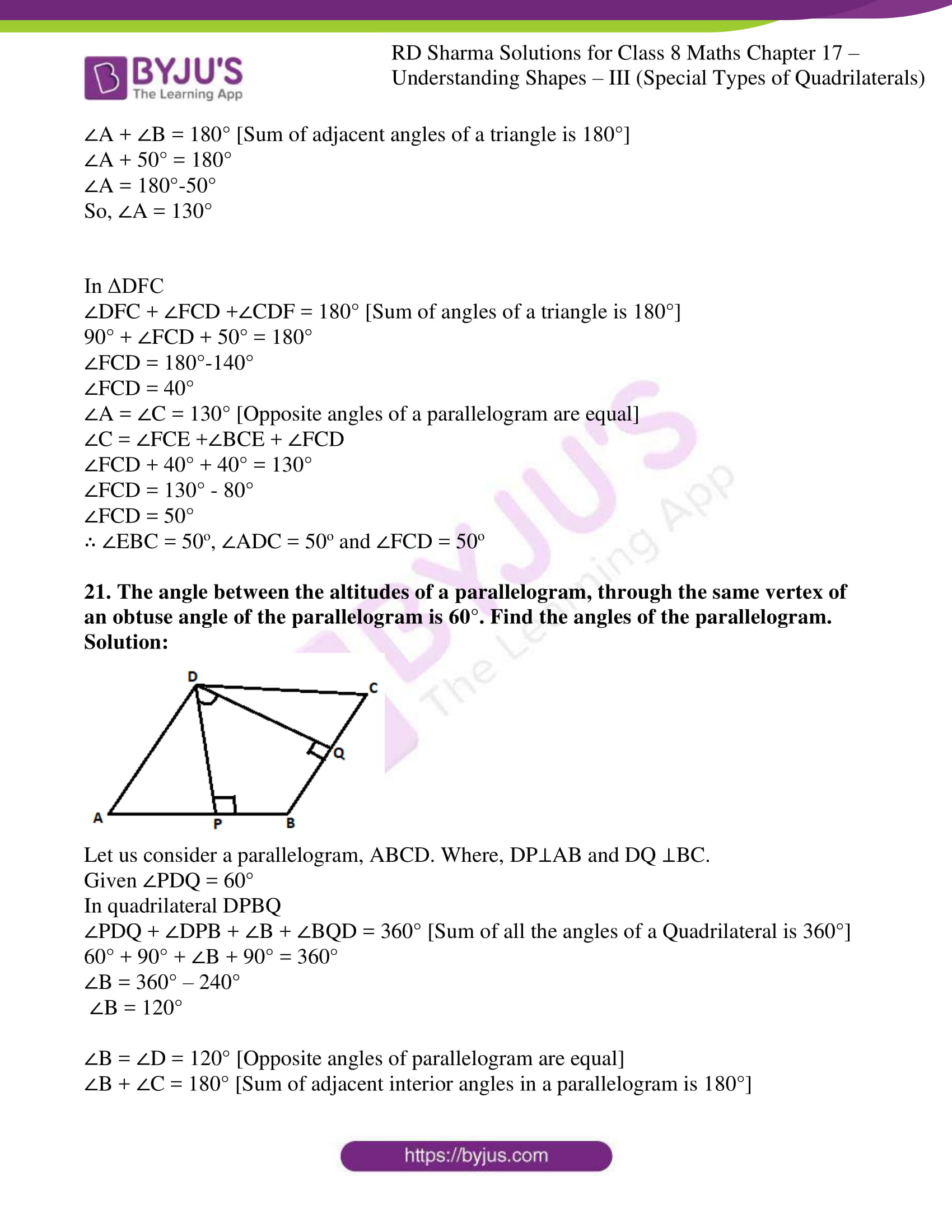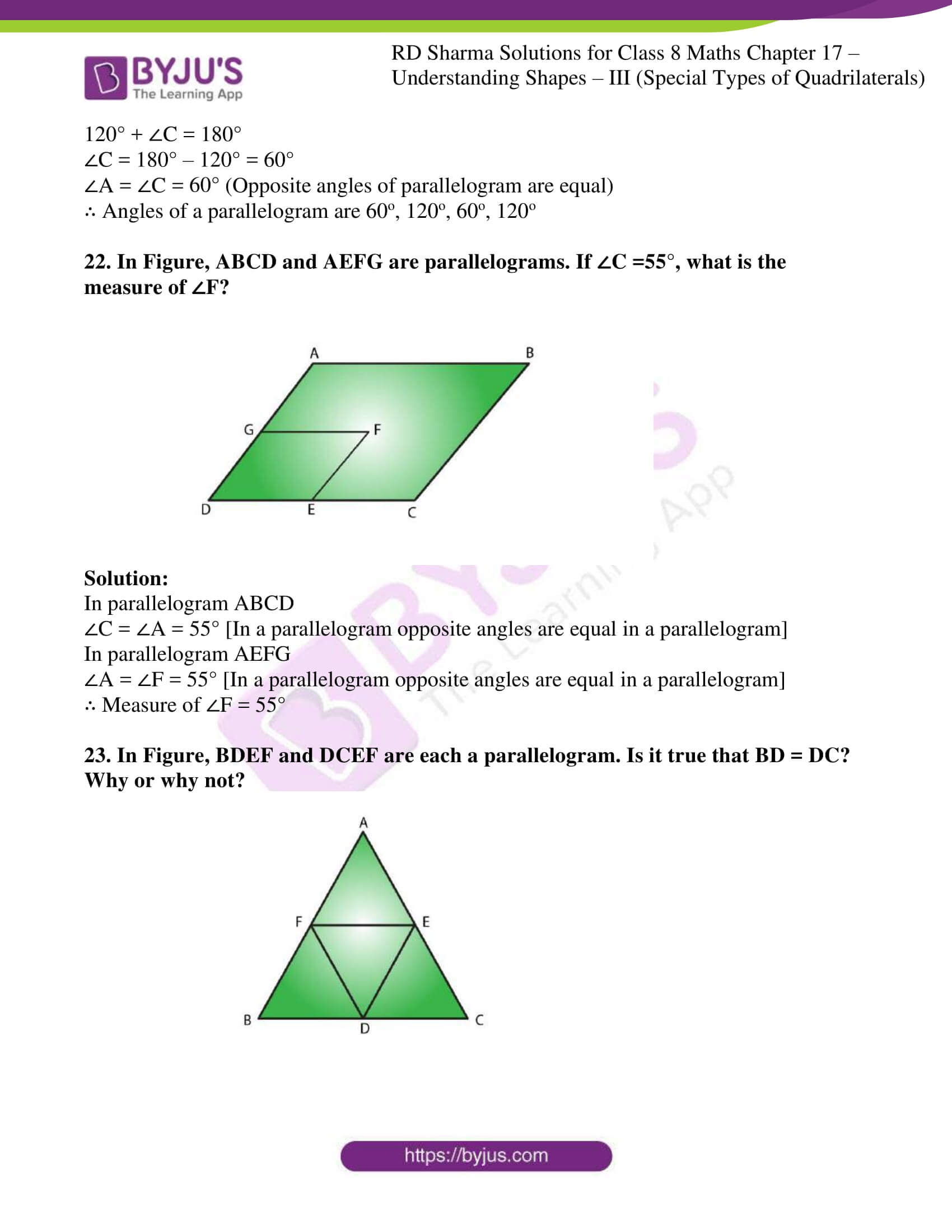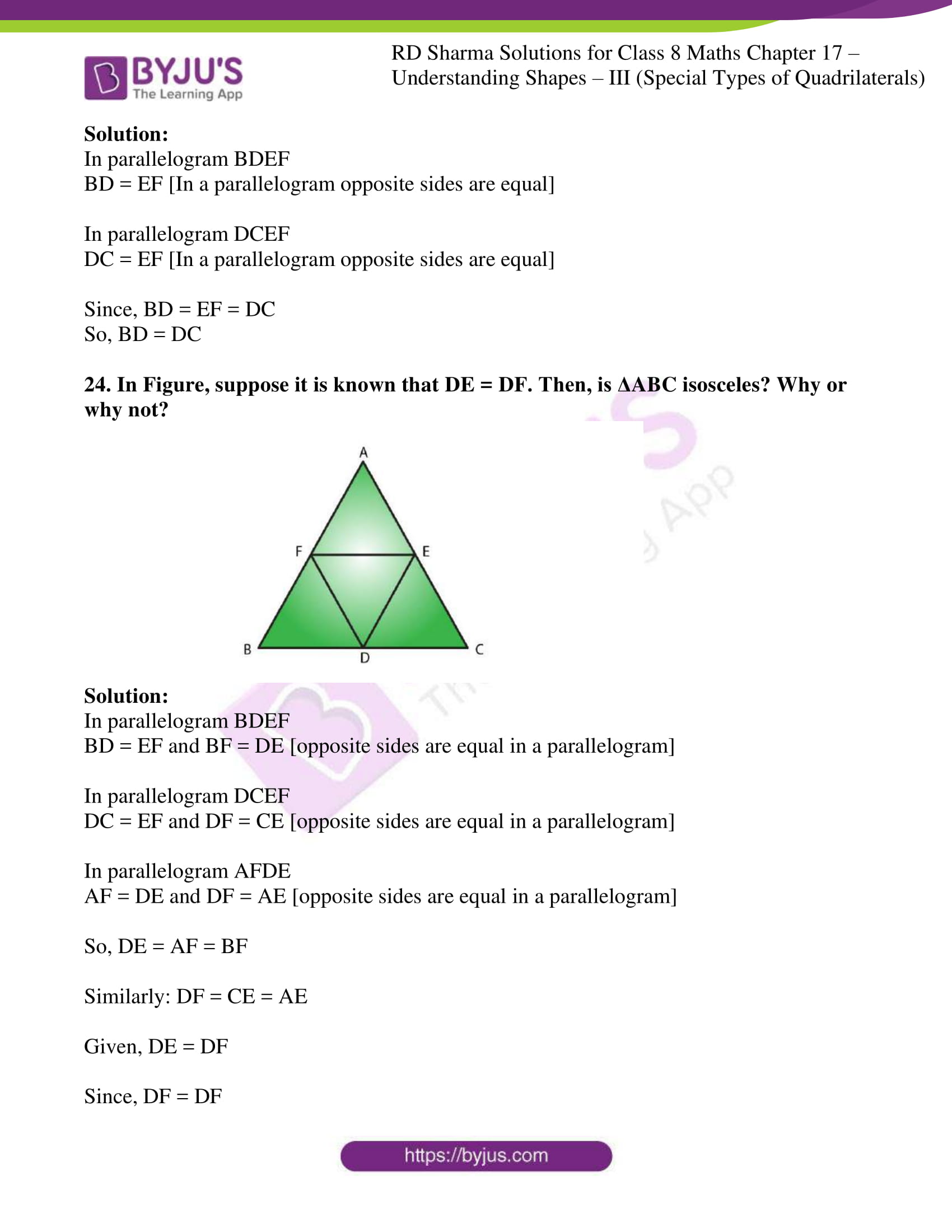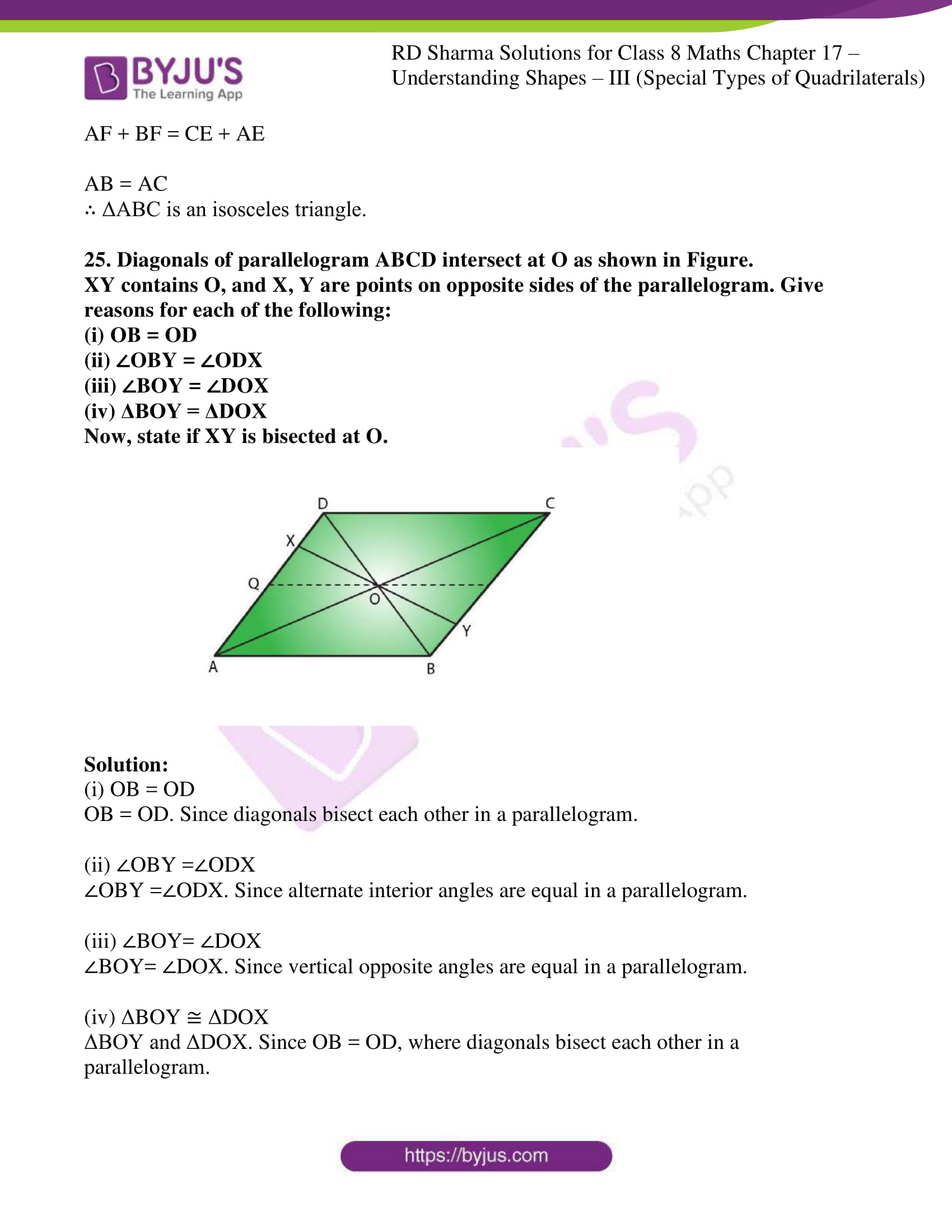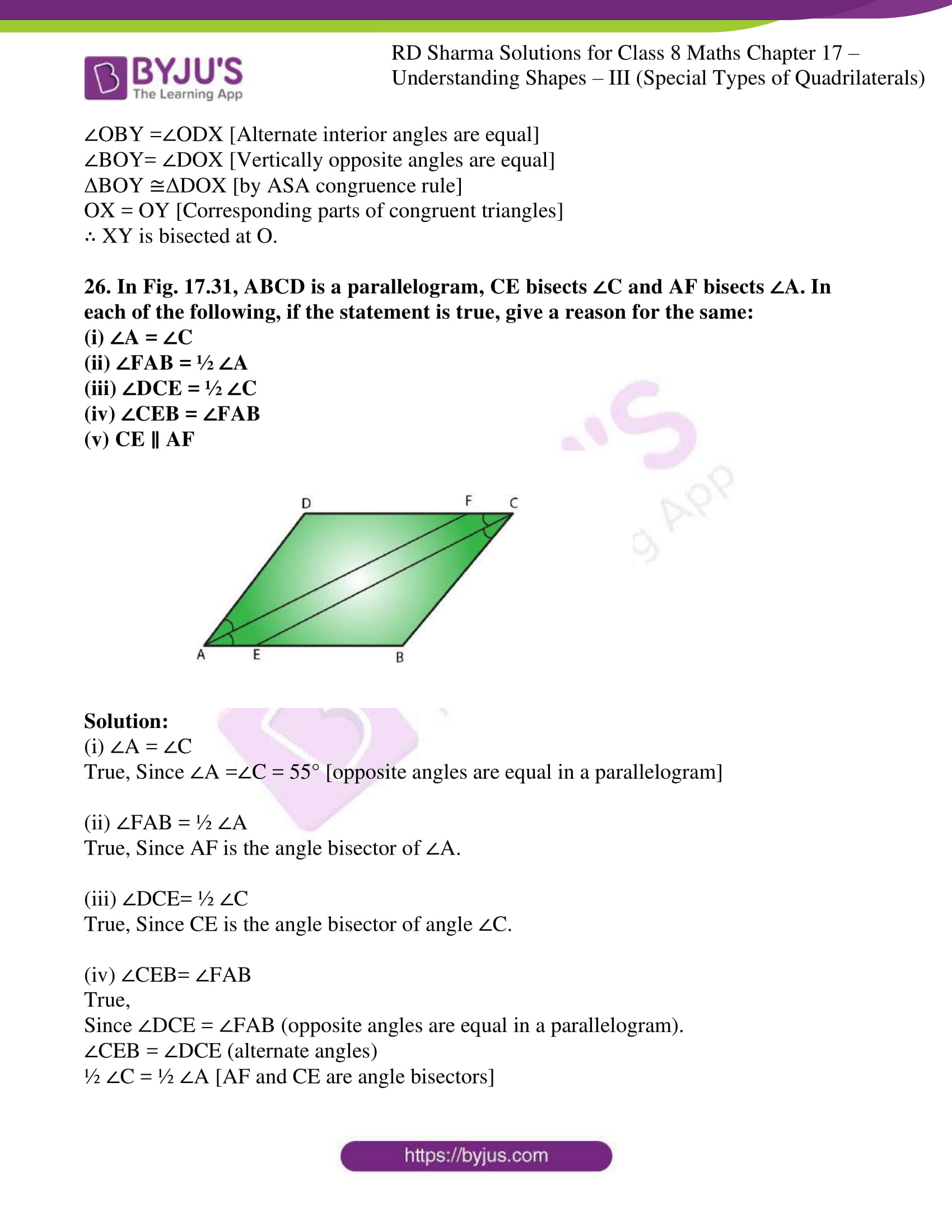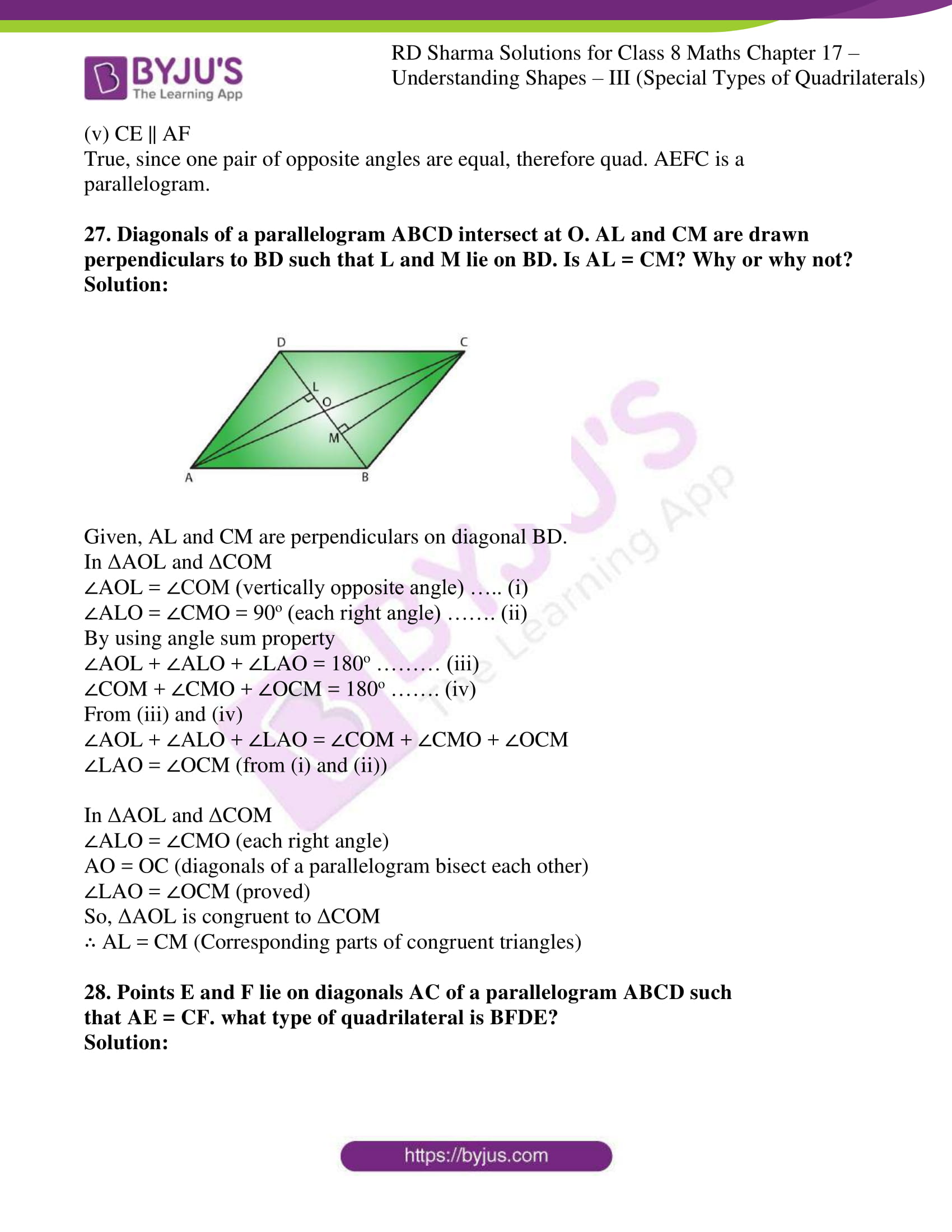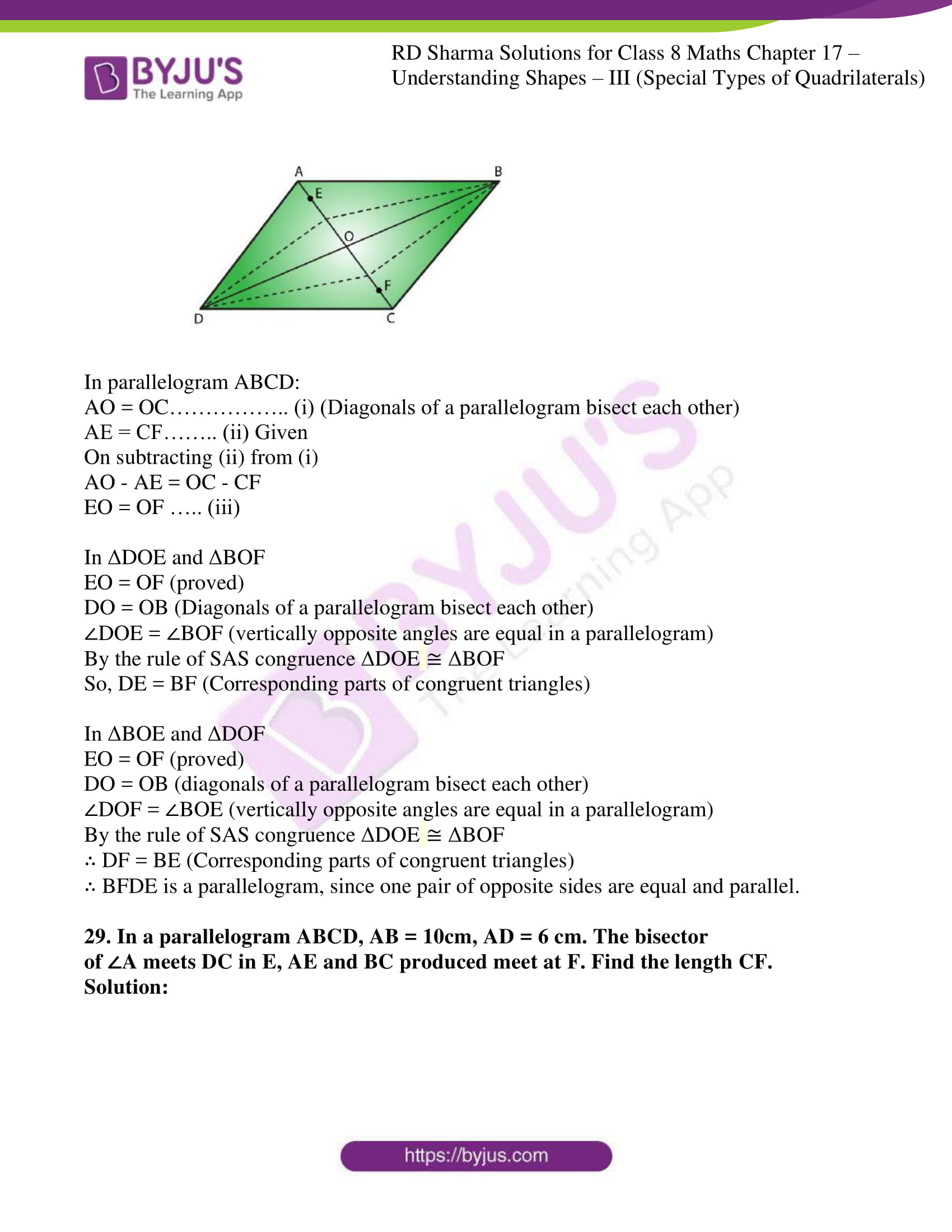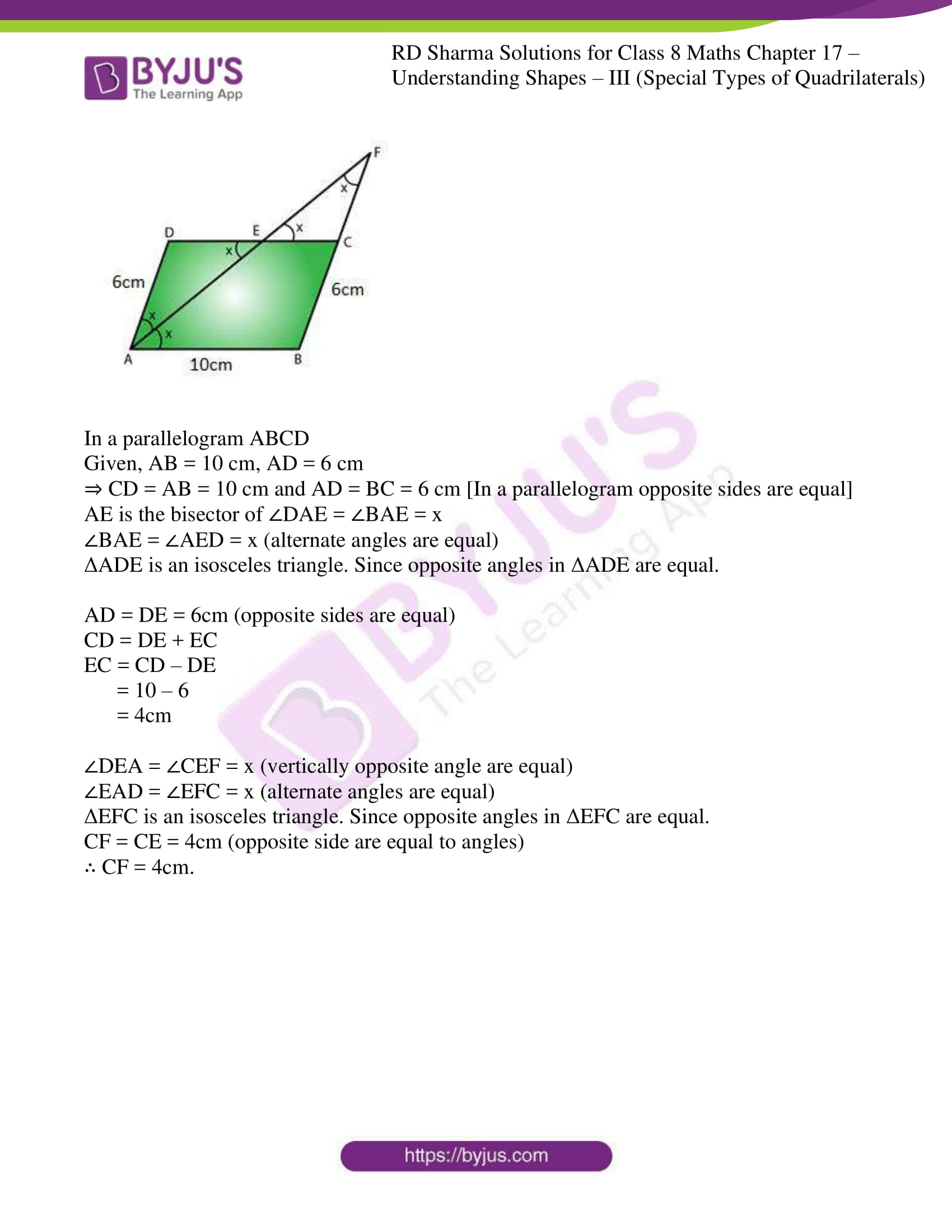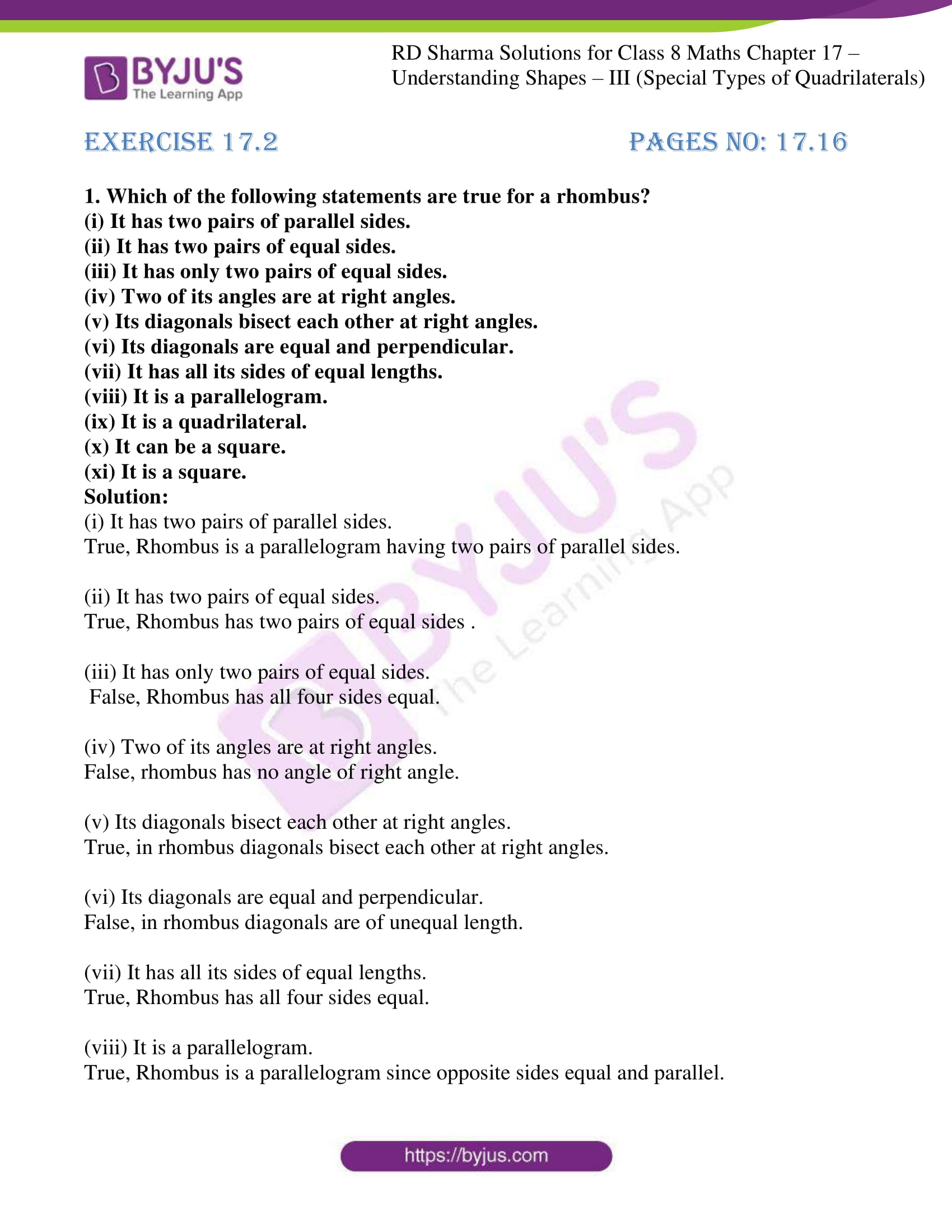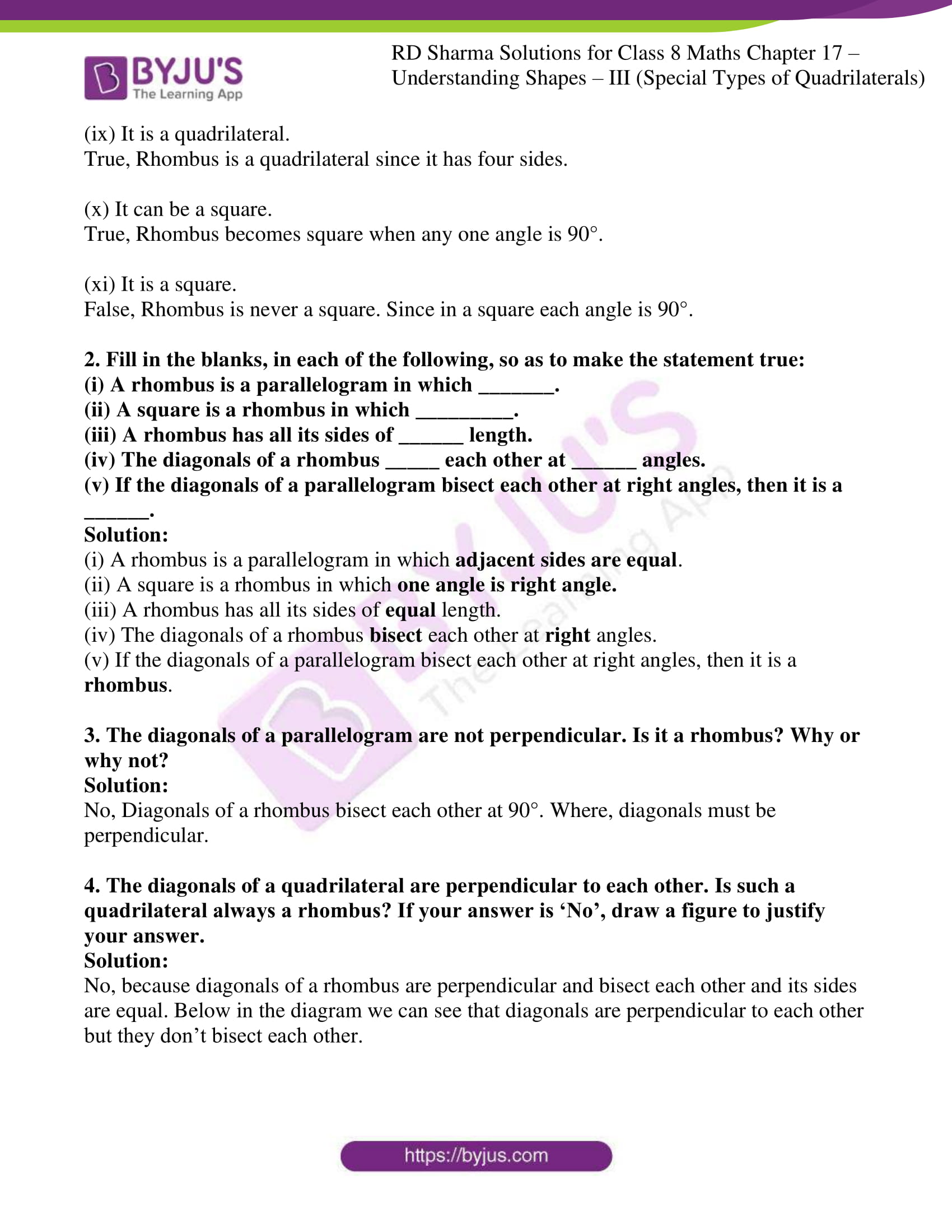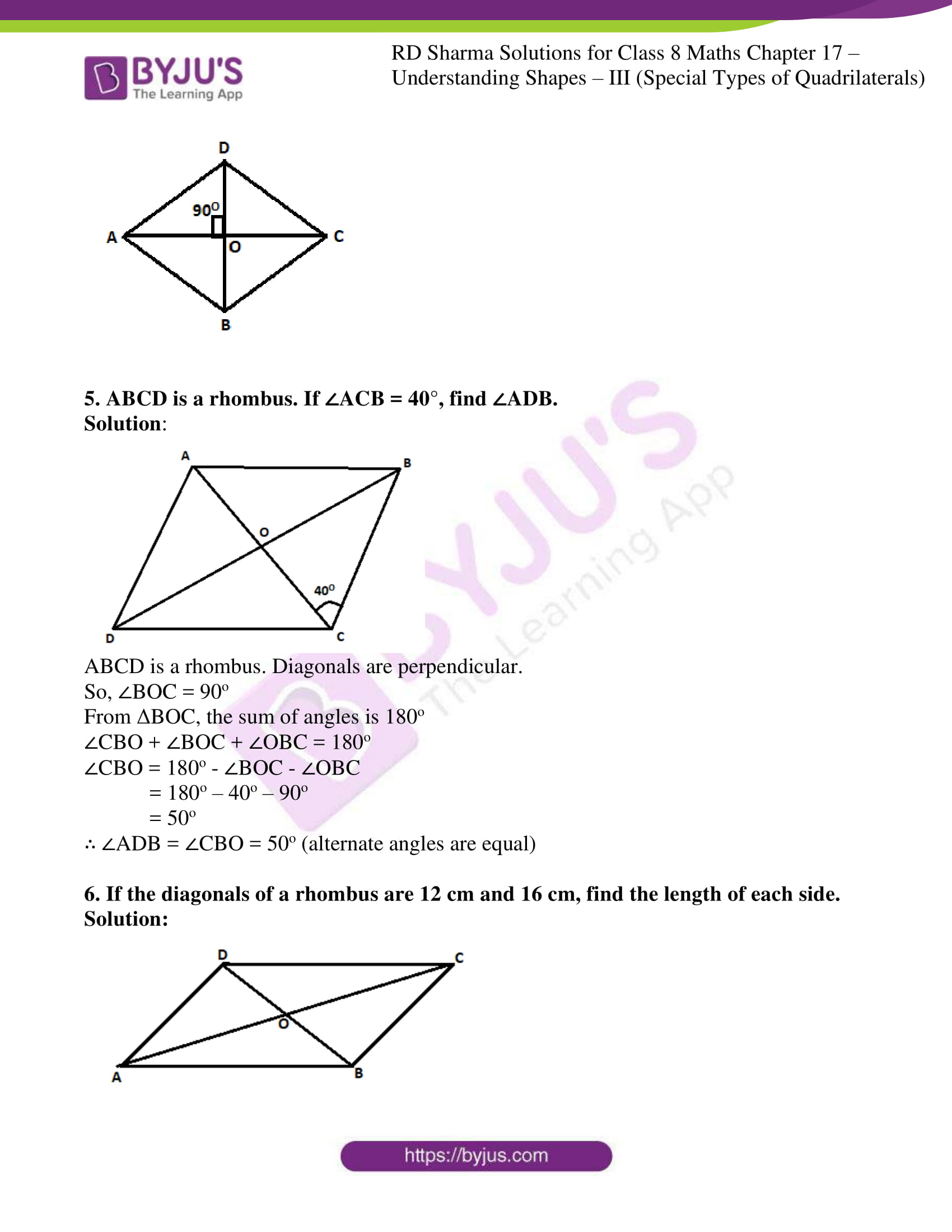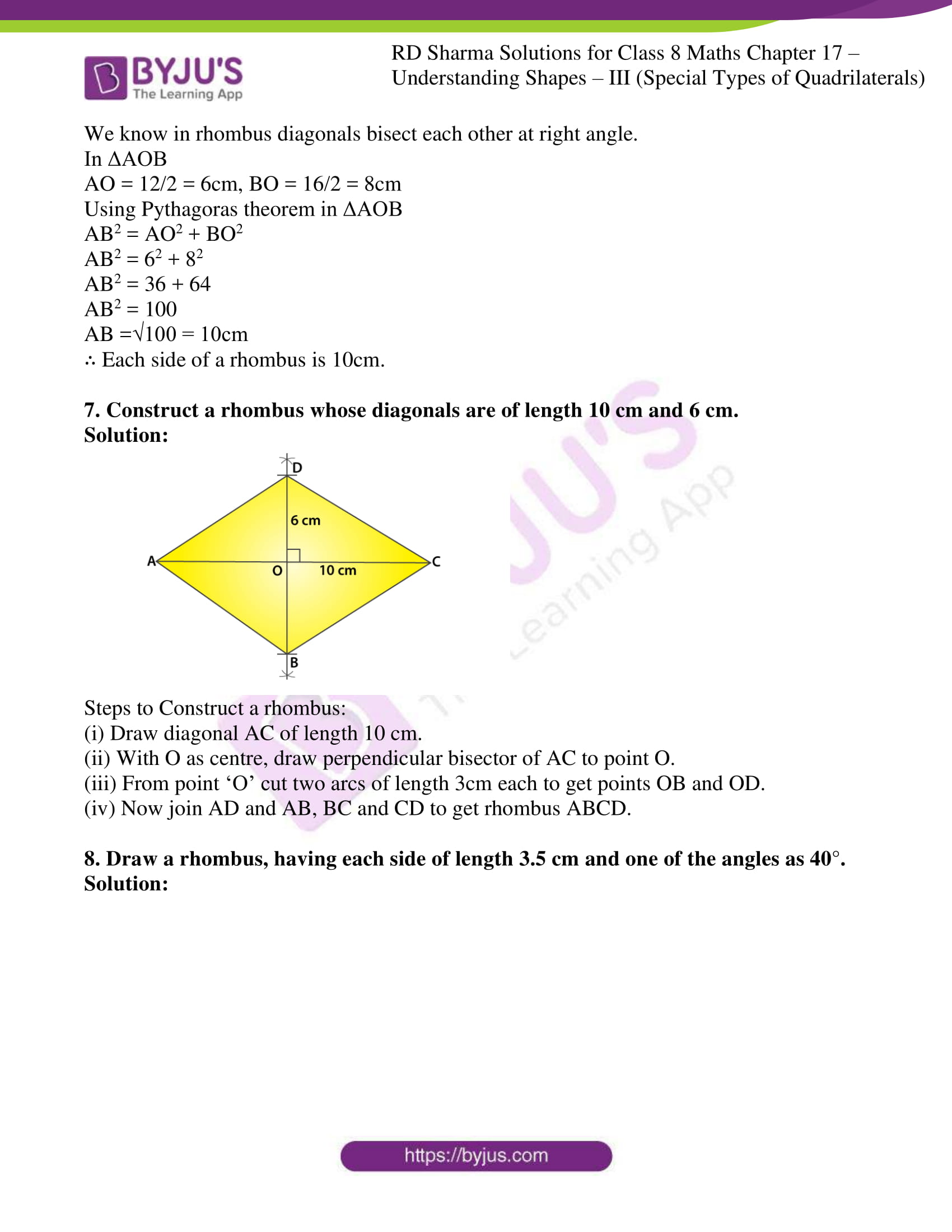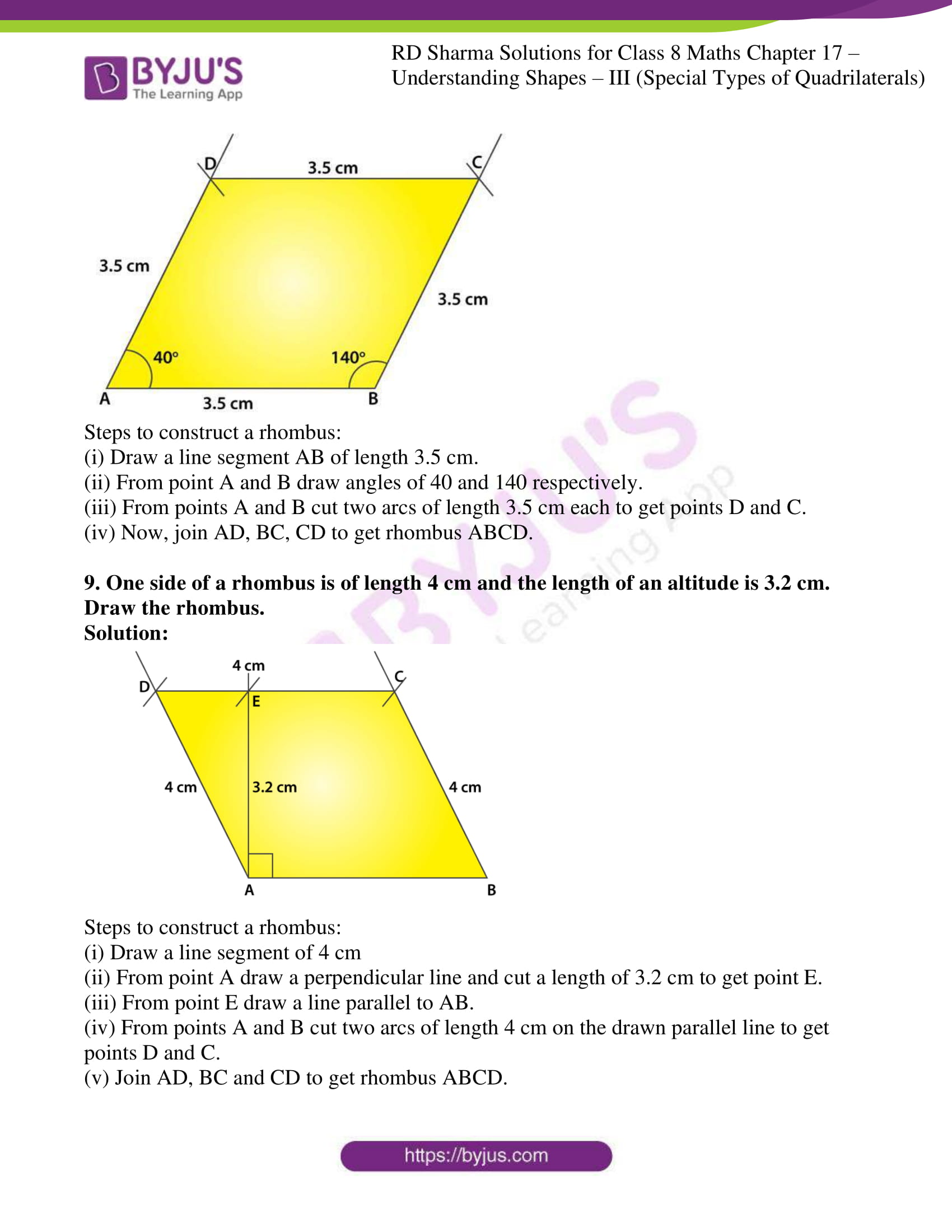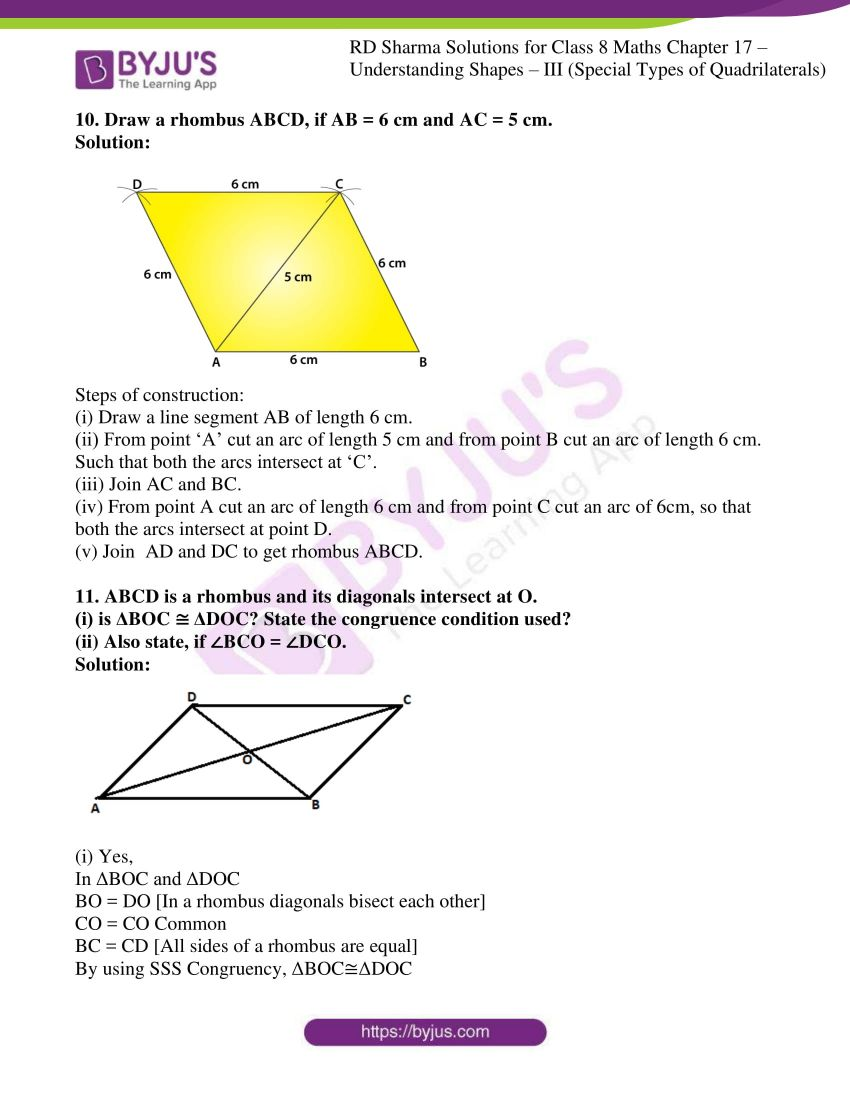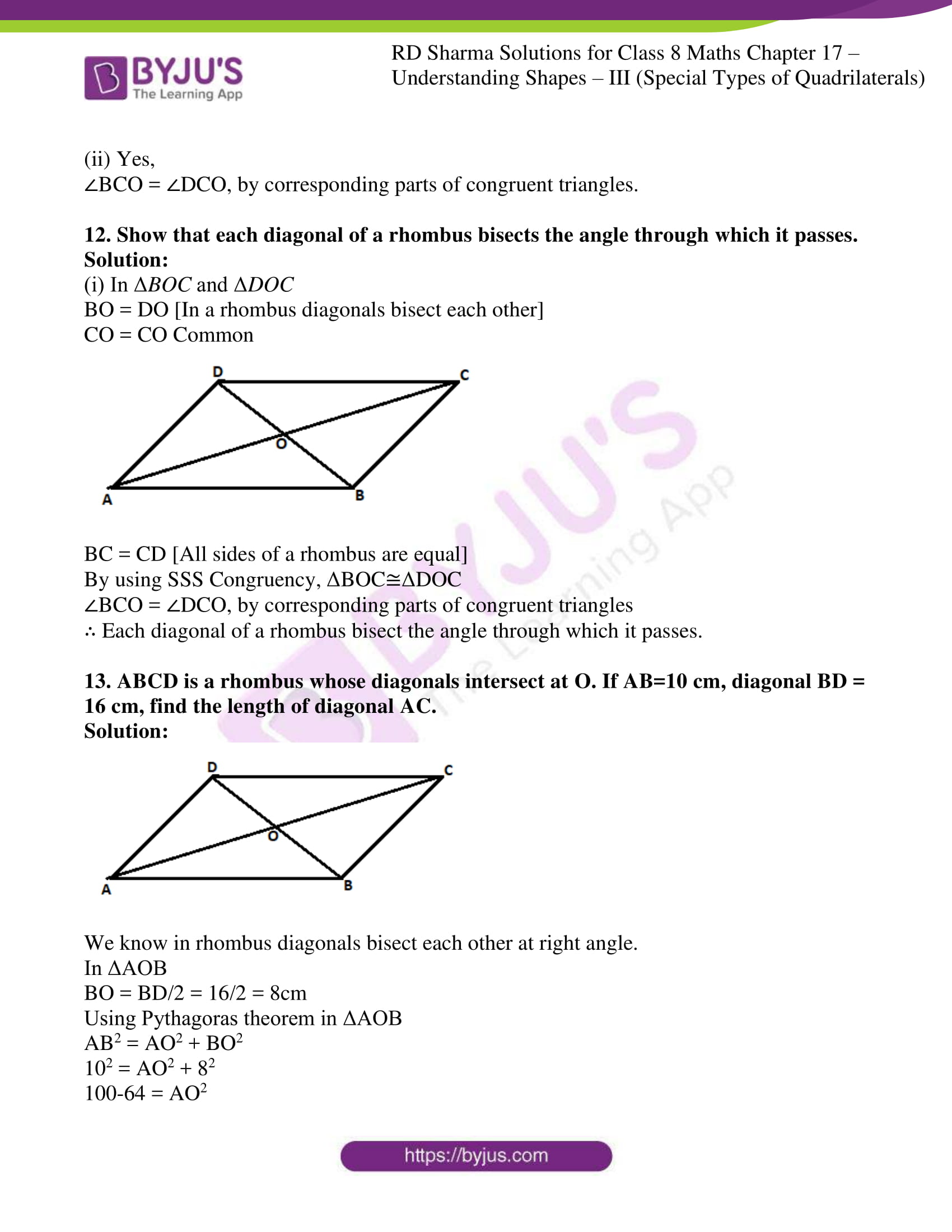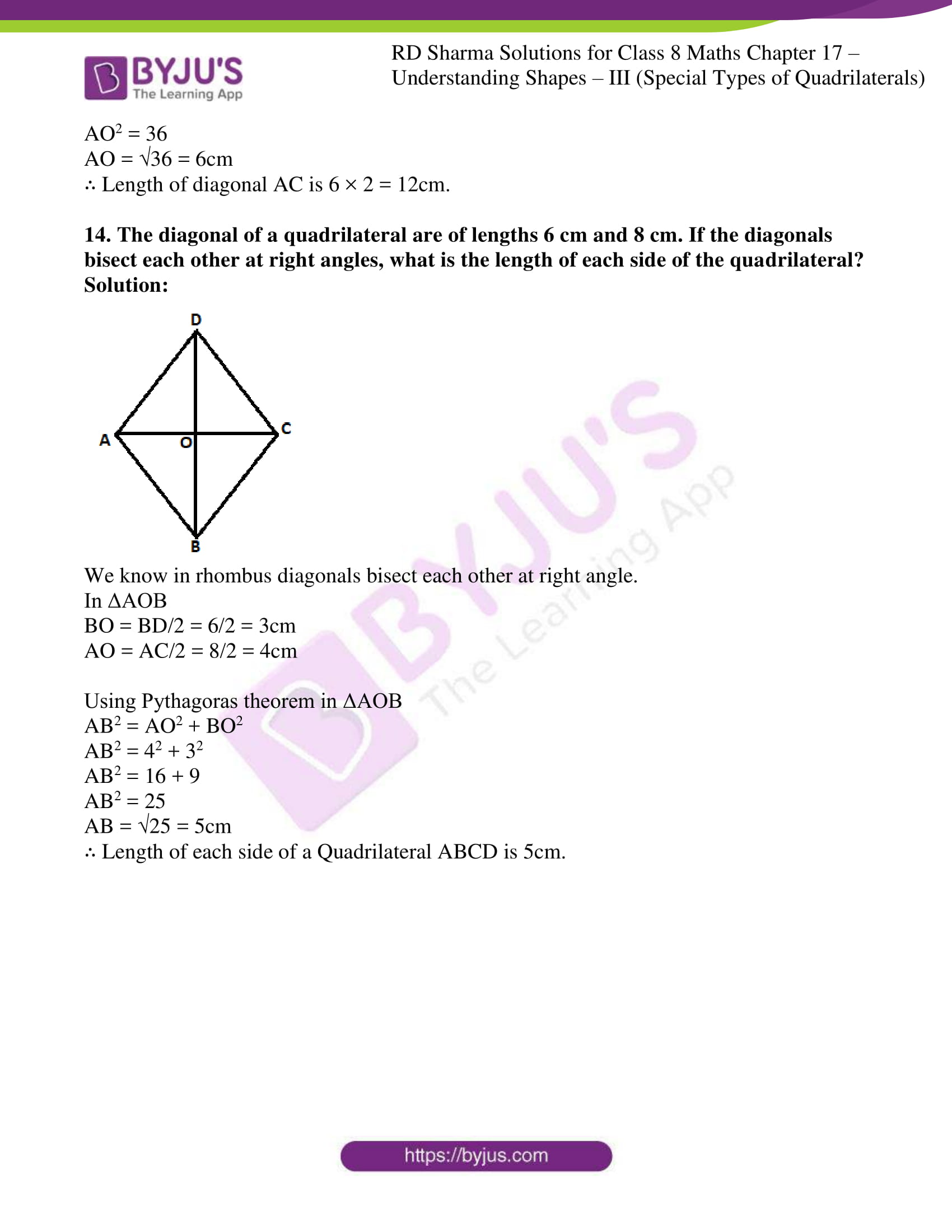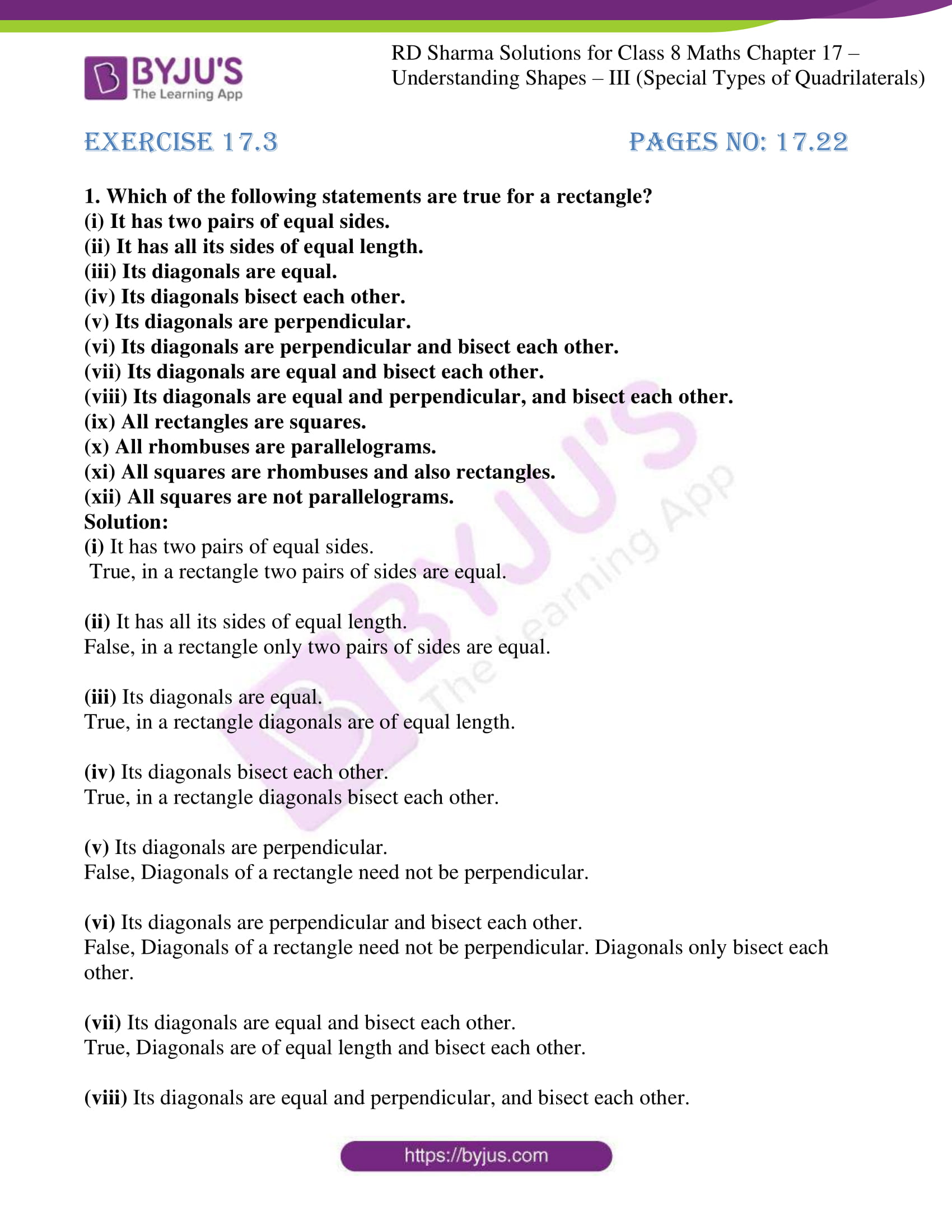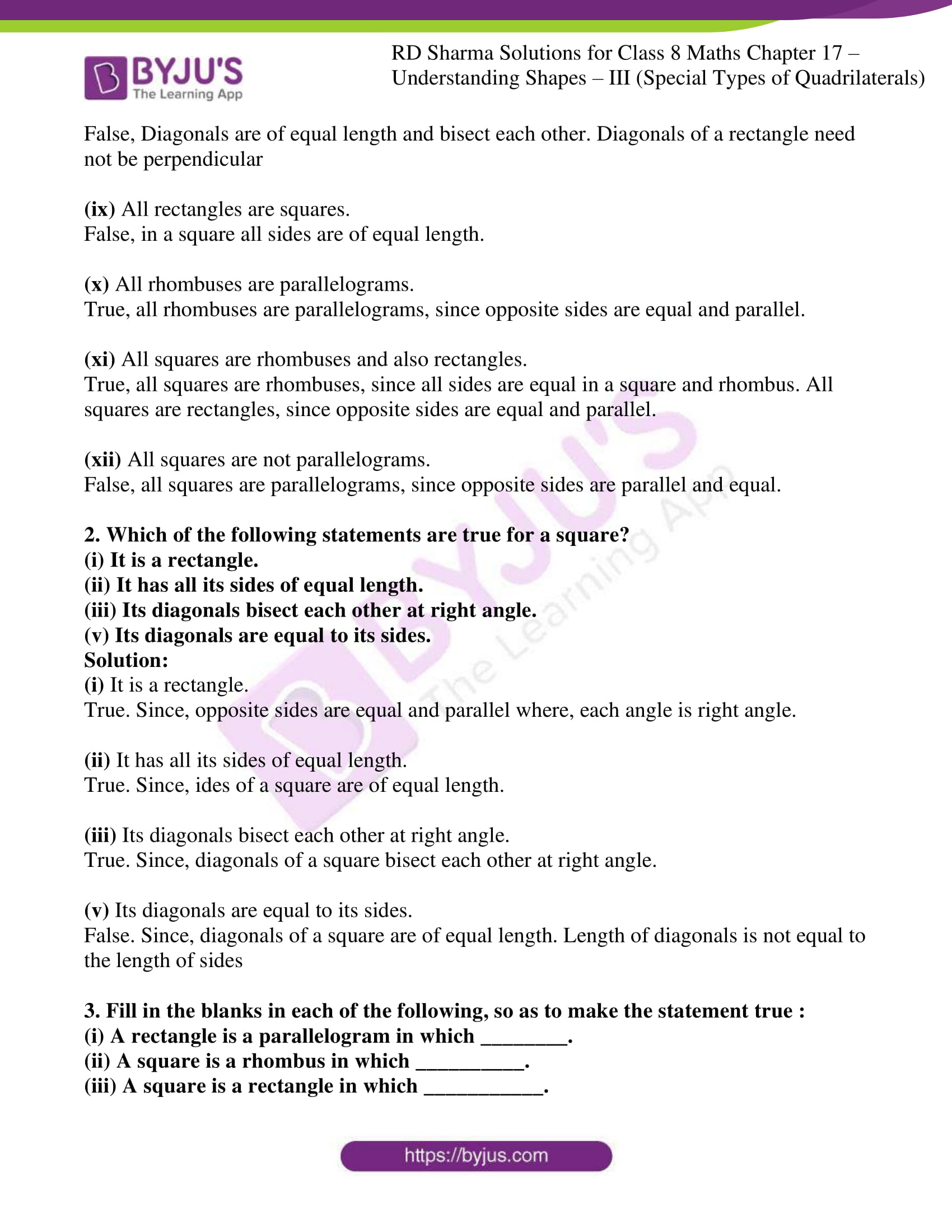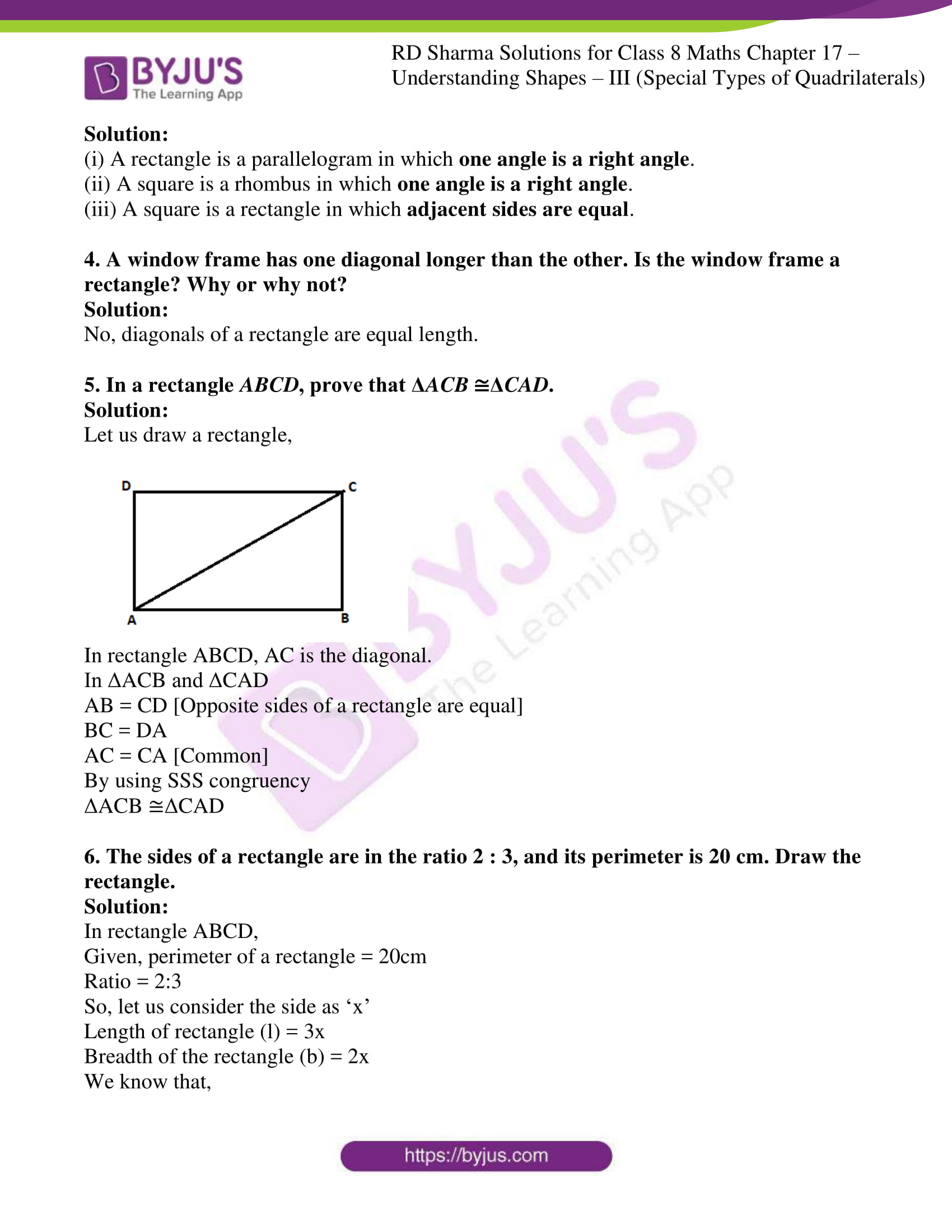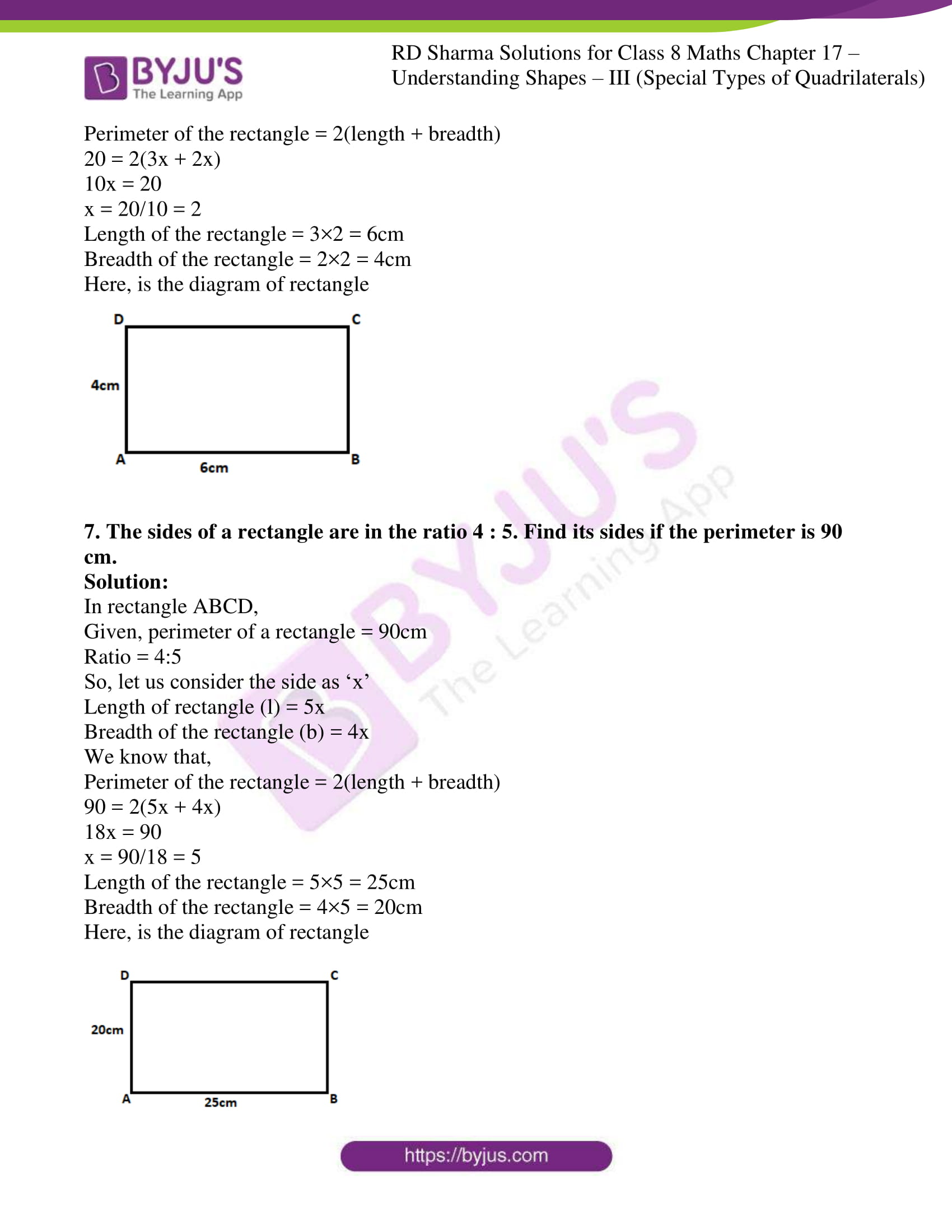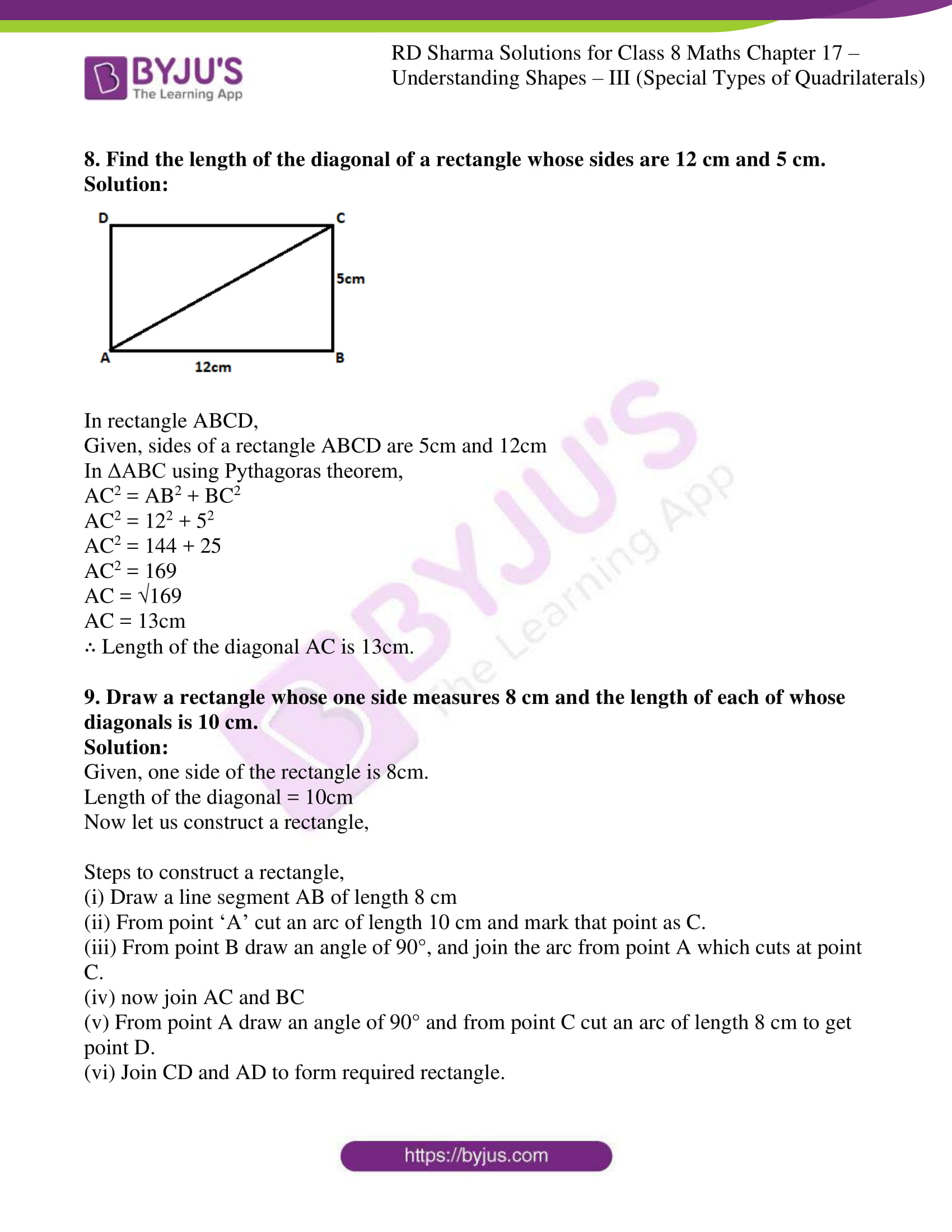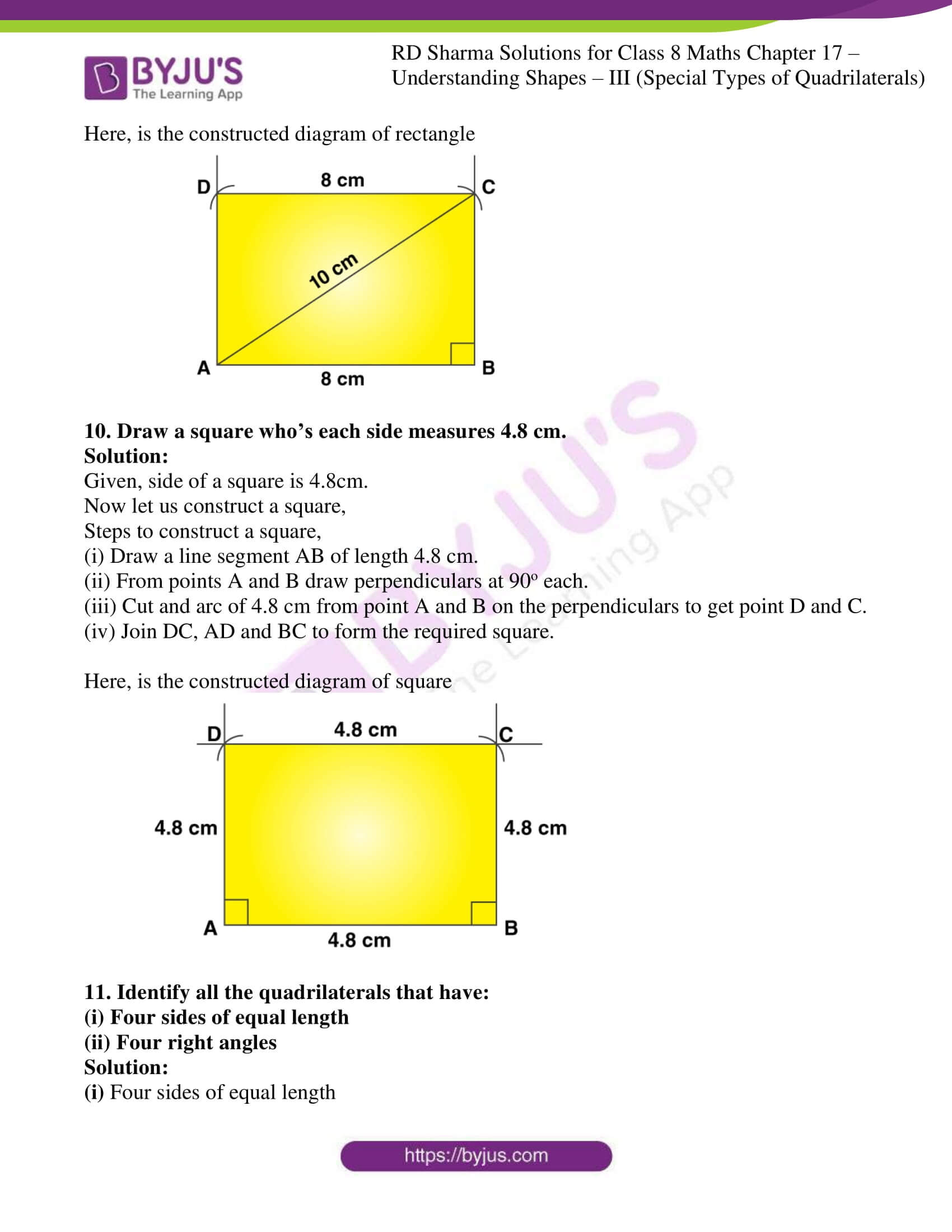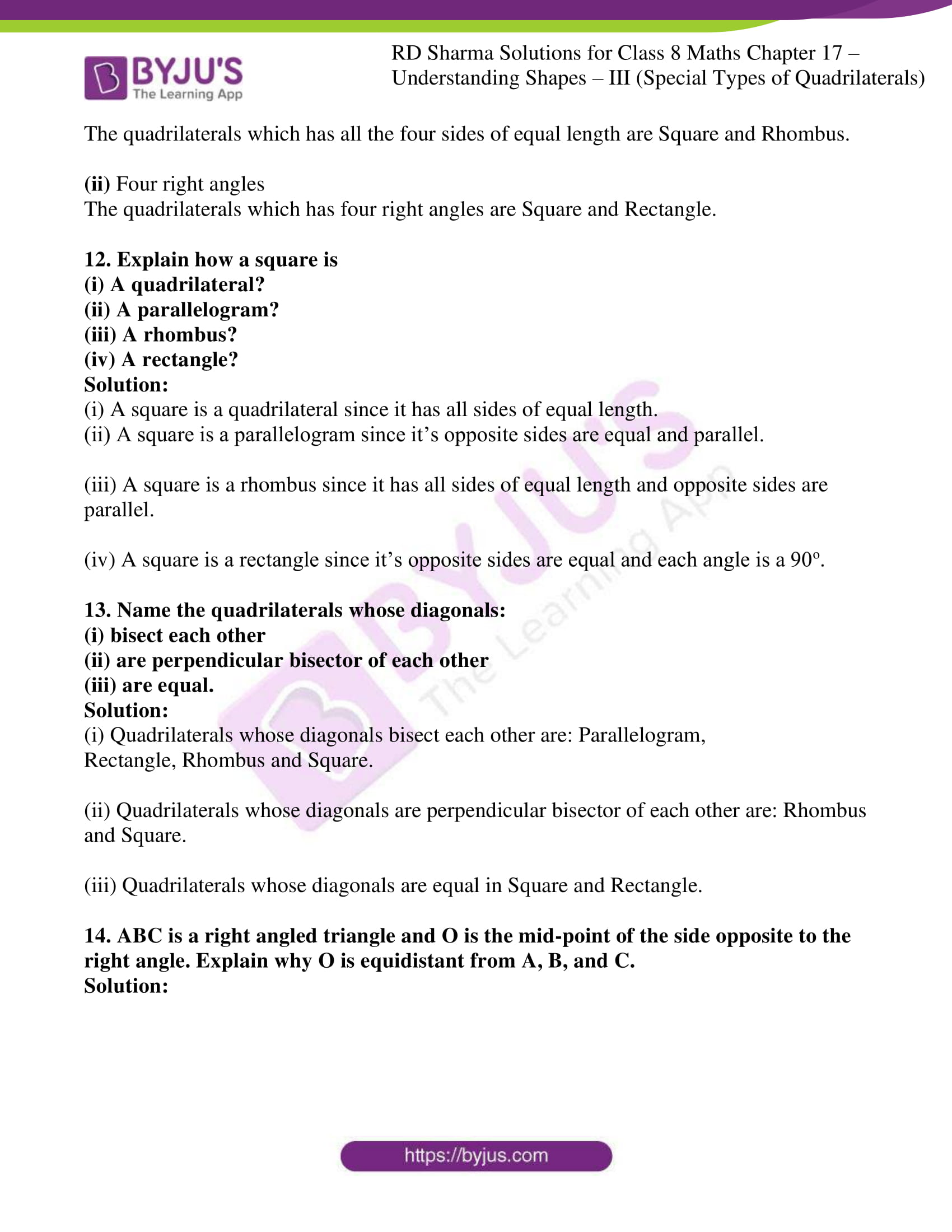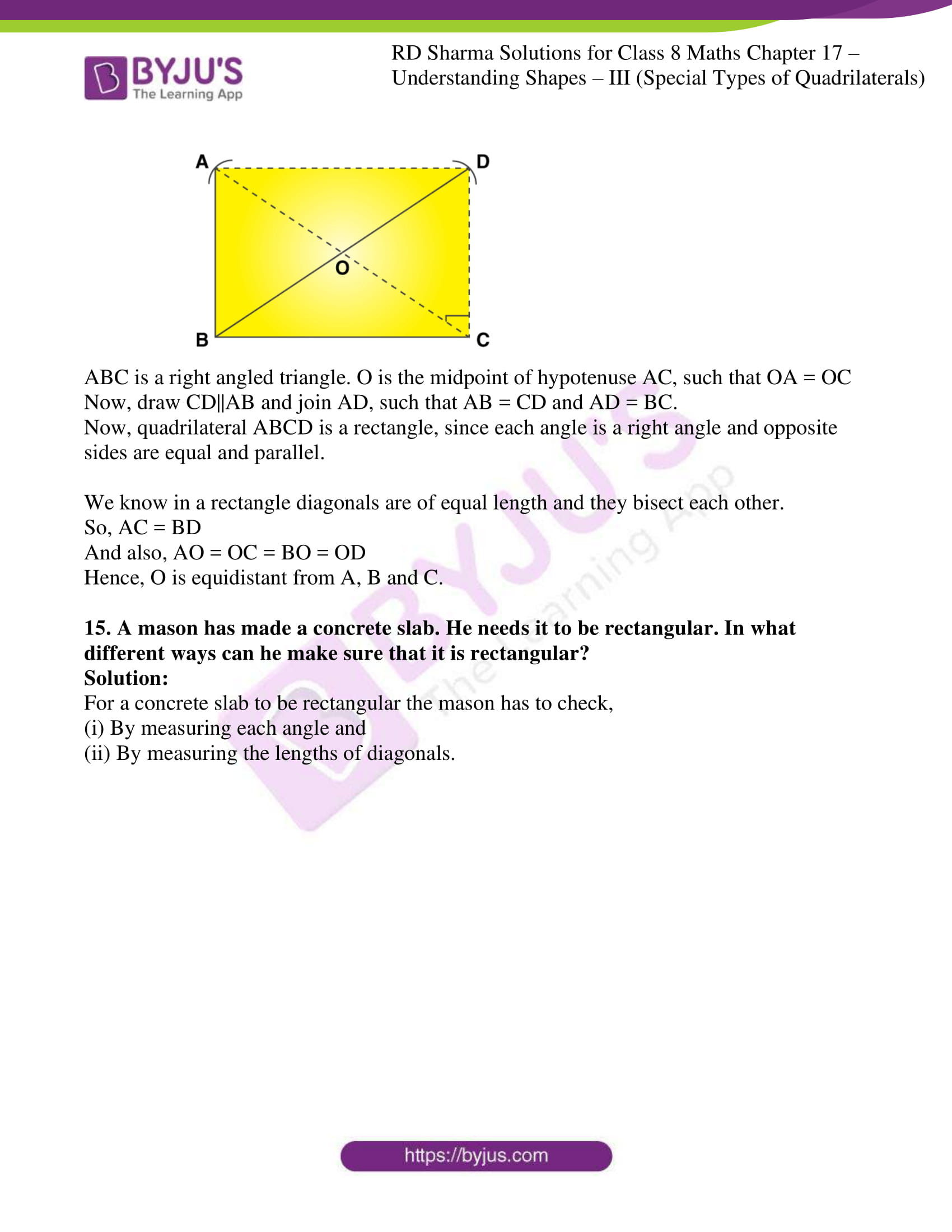## Access answers to Maths RD Sharma Solutions for Class 8 Chapter 17 – Understanding Shapes – III (Special Types of Quadrilaterals)

EXERCISE 17.1 PAGE NO: 17.9

1. Given below is a parallelogram ABCD. Complete each statement along with the definition or property used.

(ii) ∠DCB =
(iii) OC =

(iv) ∠DAB + ∠CDA =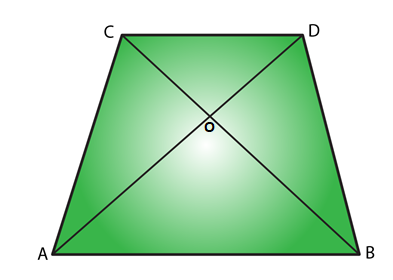Solution:

(i) AD = BC. Because, diagonals bisect each other in a parallelogram.

(ii) ∠DCB = ∠BAD. Because, alternate interior angles are equal.

(iii) OC = OA. Because, diagonals bisect each other in a parallelogram.

(iv) ∠DAB+ ∠CDA = 180°. Because sum of adjacent angles in a parallelogram is 180°.

2. The following figures are parallelograms. Find the degree values of the unknowns x, y, z.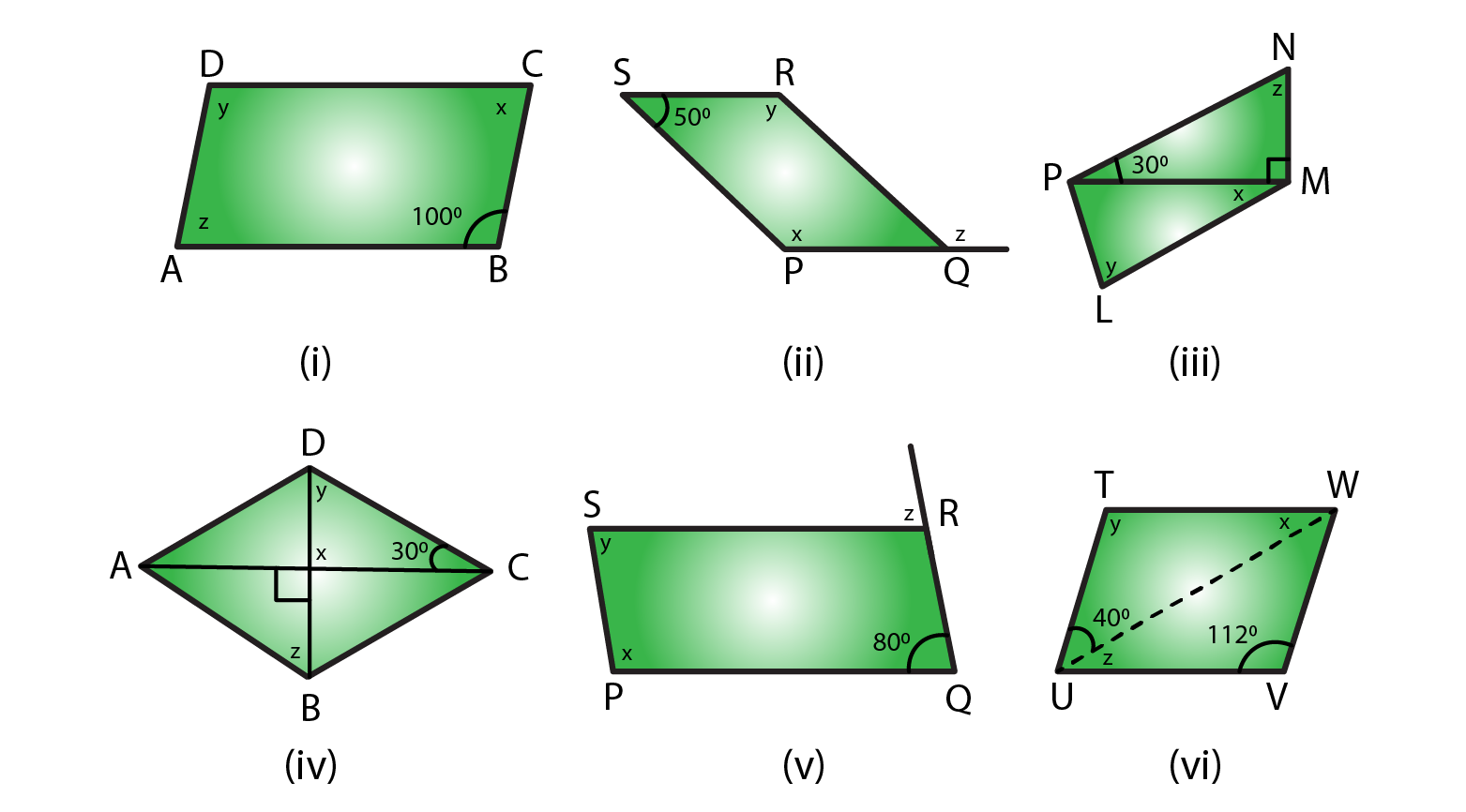Solution:

(i) ∠ABC = ∠y = 100o (opposite angles are equal in a parallelogram)

∠x + ∠y = 180o (sum of adjacent angles is = 180o in a parallelogram)

∠x + 100o = 180o

∠x = 180o – 100o

= 80o

∴ ∠x = 80o ∠y = 100o∠z = 80o (opposite angles are equal in a parallelogram)

(ii) ∠RSP + ∠y = 180o (sum of adjacent angles is = 180o in a parallelogram)

∠y + 50o = 180o

∠y = 180o – 50o

= 130o

∴ ∠x = ∠y = 130o (opposite angles are equal in a parallelogram)

∠RSP = ∠RQP = 50o (opposite angles are equal in a parallelogram)

∠RQP + ∠z = 180o (linear pair)

50o + ∠z = 180o

∠z = 180o – 50o

= 130o

∴ ∠x = 130o ∠y = 130o ∠z = 130o

(iii) In ΔPMN

∠NPM + ∠NMP + ∠MNP = 180° [Sum of all the angles of a triangle is 180°]

30° + 90° + ∠z = 180°

∠z = 180°-120°

= 60°

∠y = ∠z = 60° [opposite angles are equal in a parallelogram]

∠z = 180°-120° [sum of the adjacent angles is equal to 180° in a parallelogram]

∠z = 60°

∠z + ∠LMN = 180° [sum of the adjacent angles is equal to 180° in a parallelogram]

60° + 90°+ ∠x = 180°

∠x = 180°-150°

∠x = 30°

∴ ∠x = 30o ∠y = 60o ∠z = 60o

(iv) ∠x = 90° [vertically opposite angles are equal]

In ΔDOC

∠x + ∠y + 30° = 180° [Sum of all the angles of a triangle is 180°]

90° + 30° + ∠y = 180°

∠y = 180°-120°

∠y = 60°

∠y = ∠z = 60° [alternate interior angles are equal]

∴ ∠x = 90o ∠y = 60o ∠z = 60o

(v) ∠x + ∠POR = 180° [sum of the adjacent angles is equal to 180° in a parallelogram]

∠x + 80° = 180°

∠x = 180°-80°

∠x = 100°

∠y = 80° [opposite angles are equal in a parallelogram]

∠SRQ =∠x = 100°

∠SRQ + ∠z = 180° [Linear pair]

100° + ∠z = 180°

∠z = 180°-100°

∠z = 80°

∴ ∠x = 100o ∠y = 80o ∠z = 80o

(vi) ∠y = 112° [In a parallelogram opposite angles are equal]

∠y + ∠VUT = 180° [In a parallelogram sum of the adjacent angles is equal to 180°]

∠z + 40° + 112° = 180°

∠z = 180°-152°

∠z = 28°

∠z =∠x = 28° [alternate interior angles are equal]

∴ ∠x = 28o ∠y = 112o ∠z = 28o

3. Can the following figures be parallelograms? Justify your answer.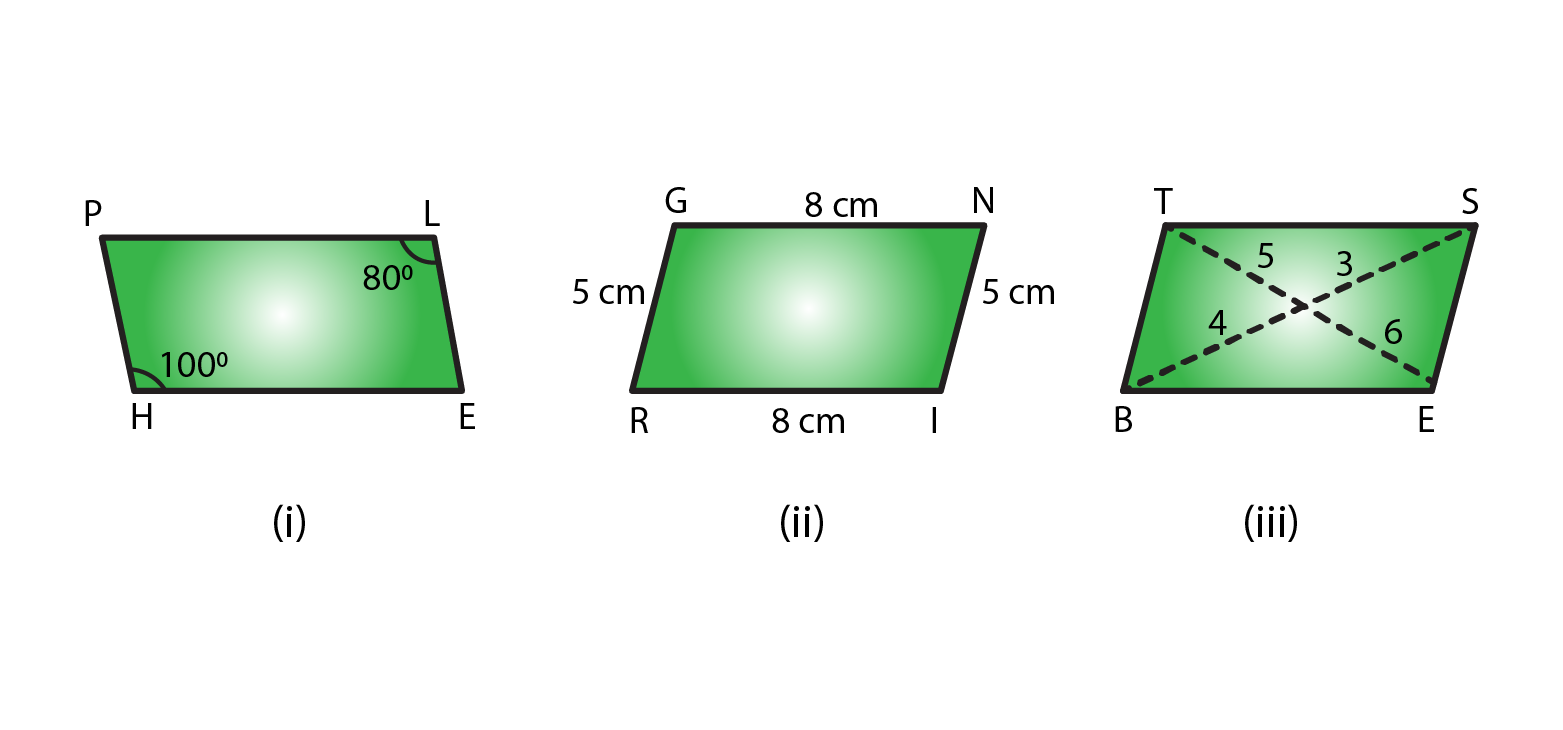Solution:

(i) No, opposite angles are equal in a parallelogram.

(ii) Yes, opposite sides are equal and parallel in a parallelogram.

(iii) No, diagonals bisect each other in a parallelogram.

4. In the adjacent figure HOPE is a parallelogram. Find the angle measures x, y and z. State the geometrical truths you use to find them.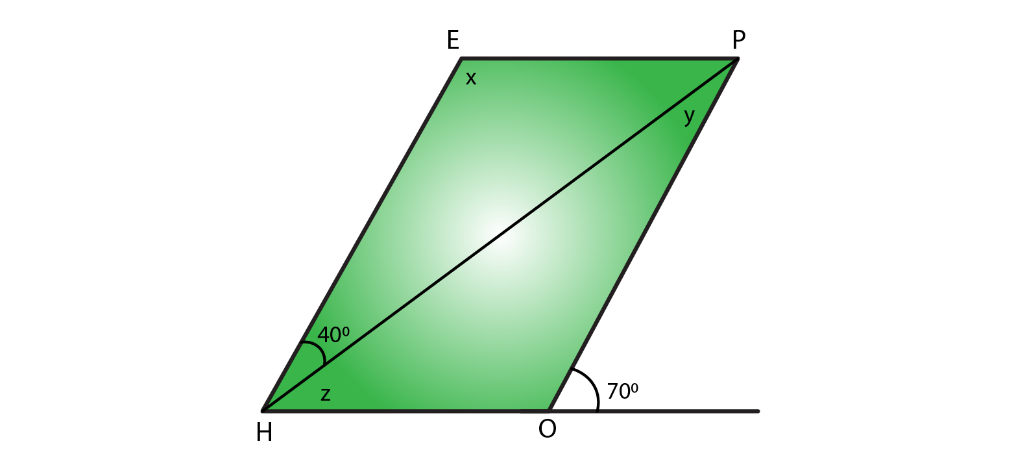Solution:

We know that

∠POH + 70° = 180° [Linear pair]

∠POH = 180°-70°

∠POH = 110°

∠POH = ∠x = 110° [opposite angles are equal in a parallelogram]

∠x + ∠z + 40° = 180° [sum of the adjacent angles is equal to 180° in a parallelogram]

110° + ∠z + 40° = 180°

∠z = 180° – 150°

∠z = 30°

∠z +∠y = 70°

∠y + 30° = 70°

∠y = 70°- 30°

∠y = 40°

5. In the following figures GUNS and RUNS are parallelograms. Find x and y.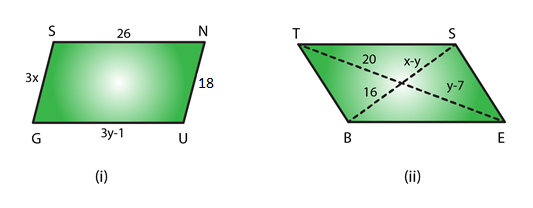Solution:

(i) 3y – 1 = 26 [opposite sides are of equal length in a parallelogram]

3y = 26 + 1

y = 27/3

y = 9

3x = 18 [opposite sides are of equal length in a parallelogram]

x = 18/3

x = 6

∴ x = 6 and y = 9

(ii) y – 7 = 20 [diagonals bisect each other in a parallelogram]

y = 20 + 7

y = 27

x – y = 16 [diagonals bisect each other in a parallelogram]

x -27 = 16

x = 16 + 27

= 43

∴ x = 43 and y = 27

6. In the following figure RISK and CLUE are parallelograms. Find the measure of x.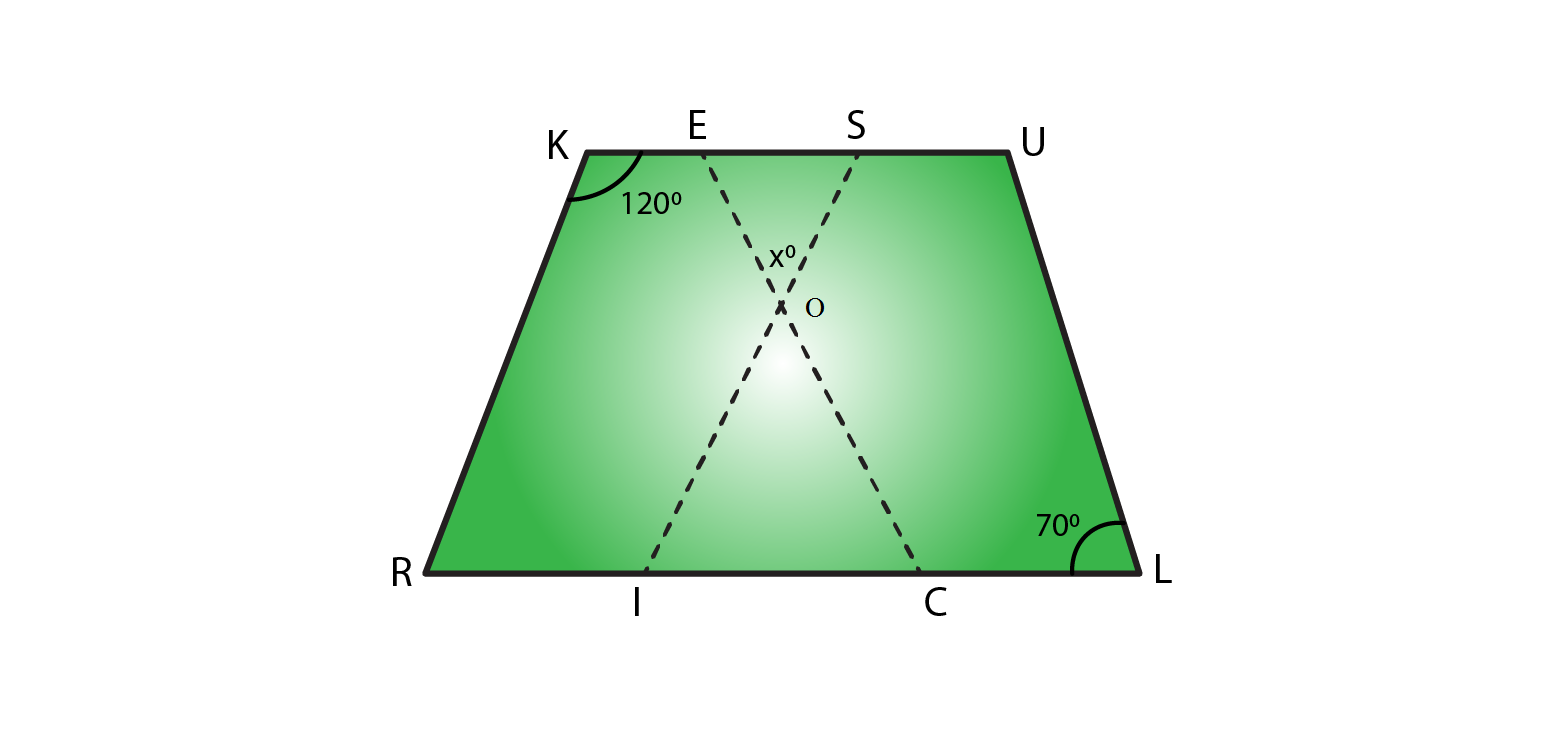Solution:

In parallelogram RISK

∠RKS + ∠KSI = 180° [sum of the adjacent angles is equal to 180° in a parallelogram]

120° + ∠KSI = 180°

∠KSI = 180° – 120°

∠KSI = 60°

In parallelogram CLUE

∠CEU = ∠CLU = 70° [opposite angles are equal in a parallelogram]

In ΔEOS

70° + ∠x + 60° = 180° [Sum of angles of a triangles is 180°]

∠x = 180° – 130°

∠x = 50°

∴ x = 50°

7. Two opposite angles of a parallelogram are (3– 2)o and (50 – x)o. Find the measure of each angle of the parallelogram.

Solution:

We know that opposite angles of a parallelogram are equal.

So, (3x – 2)° = (50 – x)°

3xo – 2° = 50° – xo

3x° + xo = 50° + 2°

4xo = 52°

xo = 52o/4

= 13o

Measure of opposite angles are,

(3x – 2)o = 3×13 – 2 = 37°

(50 – x)o = 50 – 13 = 37°

We know that Sum of adjacent angles = 180°

Other two angles are 180° – 37° = 143°

∴ Measure of each angle is 37o, 143o, 37o, 143o

8. If an angle of a parallelogram is two-third of its adjacent angle, find the angles of the parallelogram.

Solution:

Let us consider one of the adjacent angle as x°

Other adjacent angle is = 2xo/3

We know that sum of adjacent angles = 180°

So,

xo + 2xo/3 = 180o

(3xo + 2xo)/3 = 180o

5xo/3 = 180o

xo = 180o×3/5

= 108o

Other angle is = 180o – 108o = 72o

∴ Angles of a parallelogram are 72o, 72o, 108o, 108o

9. The measure of one angle of a parallelogram is 70°. What are the measures of the remaining angles?

Solution:

Let us consider one of the adjacent angle as x°

Other adjacent angle = 70°

We know that sum of adjacent angles = 180°

So,

xo + 70o = 180o

xo = 180o – 70o

= 110o

∴ Measures of the remaining angles are 70°, 70°, 110° and 110°

10. Two adjacent angles of a parallelogram are as 1 : 2. Find the measures of all the angles of the parallelogram.

Solution:

Let us consider one of the adjacent angle as x°

Other adjacent angle = 2x°

We know that sum of adjacent angles = 180°

So,

xo + 2xo = 180o

3xo = 180o

xo = 180o/3

= 60o

So other angle is 2x = 2×60 = 120o

∴ Measures of the remaining angles are 60°, 60°, 120° and 120°

11. In a parallelogram ABCD, ∠D= 135°, determine the measure of ∠A and ∠B.

Solution:

Given, one of the adjacent angle ∠D = 135°

Let other adjacent angle ∠A be = x°

We know that sum of adjacent angles = 180°

xo + 135o = 180o

xo = 180o – 135o

= 45o

∠A = xo = 45o

We know that measure of opposite angles are equal in a parallelogram.

So, ∠A = ∠C = 45o

And ∠D = ∠B = 135o

12. ABCD is a parallelogram in which ∠A = 70°. Compute ∠B, ∠C and ∠D.

Solution:

Given, one of the adjacent angle ∠A = 70o

Other adjacent angle ∠B be = xo

We know that sum of adjacent angles = 180°

xo + 70o = 180o

xo = 180o – 70o

= 110o

∠B = xo = 110o

We know that measure of opposite angles are equal in a parallelogram.

So, ∠A = ∠C = 70o

And ∠D = ∠B = 110o

13. The sum of two opposite angles of a parallelogram is 130°. Find all the angles of the parallelogram.

Solution:

Consider ABCD as a parallelogram

∠A + ∠C = 1300

Here ∠A and ∠C are opposite angles

So ∠C = 130/2 = 650

We know that sum of adjacent angles is 1800

∠B + ∠D = 1800

650 + ∠D = 1800

∠D = 1800 – 650 = 1150

∠D = ∠B = 1150 ( Opposite angles)

Therefore, ∠A = 650, ∠B = 1150, ∠C = 650 and ∠D = 1150.

14. All the angles of a quadrilateral are equal to each other. Find the measure of each. Is the quadrilateral a parallelogram? What special type of parallelogram is it?

Solution:

Let us consider each angle of a parallelogram as xo

We know that sum of angles = 360o

xo + xo + xo + xo = 360o

4 xo = 360o

xo = 360o/4

= 90o

∴ Measure of each angle is 90o

Yes, this quadrilateral is a parallelogram.

Since each angle of a parallelogram is equal to 90°, so it is a rectangle.

15. Two adjacent sides of a parallelogram are 4 cm and 3 cm respectively. Find its perimeter.

Solution:

We know that opposite sides of a parallelogram are parallel and equal.

So, Perimeter = Sum of all sides (there are 4 sides)

Perimeter = 4 + 3 + 4 + 3

= 14 cm

∴ Perimeter is 14cm.

16. The perimeter of a parallelogram is 150 cm. One of its sides is greater than the other by 25 cm. Find the length of the sides of the parallelogram.

Solution:

Given, Perimeter of the parallelogram = 150 cm

Let us consider one of the sides as = ‘x’ cm

Other side as = (x + 25) cm

We know that opposite sides of a parallelogram are parallel and equal.

So, Perimeter = Sum of all sides

x + x + 25 + x + x + 25 = 150

4x + 50 = 150

4x = 150 – 50

x = 100/4

= 25

∴ Sides of the parallelogram are (x) = 25 cm and (x+25) = 50 cm.

17. The shorter side of a parallelogram is 4.8 cm and the longer side is half as much again as the shorter side. Find the perimeter of the parallelogram.

Solution:

Given, Shorter side of the parallelogram = 4.8 cm

Longer side of the parallelogram = 4.8 + 4.8/2

= 4.8 + 2.4

= 7.2cm

We know that opposite sides of a parallelogram are parallel and equal.

So, Perimeter = Sum of all sides

Perimeter of the parallelogram = 4.8 + 7.2 + 4.8 + 7.2

= 24cm

∴ Perimeter of the parallelogram is 24 cm.

18. Two adjacent angles of a parallelogram are (3x-4)o and (3x+10)o. Find the angles of the parallelogram.

Solution:

We know that adjacent angles of a parallelogram are equal.

So, (3x – 4)° + (3x + 10)° = 180o

3x° + 3xo – 4 + 10 = 180°

6x = 180° – 6o

x = 174o/6

= 29o

Measure of adjacent angles are,

(3x – 4)o = 3×29 – 4 = 83°

(3x + 10)o = 3×29 + 10 = 97°

We know that Sum of adjacent angles = 180°

∴ Measure of each angle is 83o, 97o, 83o, 97o

19. In a parallelogram ABCD, the diagonals bisect each other at O.  If ∠ABC =30°, ∠BDC= 10° and ∠CAB =70°. Find:
∠DAB, ∠ADC, ∠BCD, ∠AOD, ∠DOC, ∠BOC, ∠AOB, ∠ACD, ∠CAB, ∠ADB, ∠ACB, ∠DBC, and ∠DBA.

Solution:

Firstly let us draw a parallelogram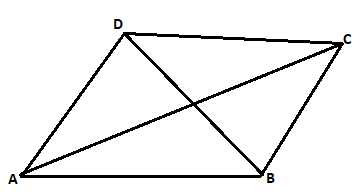Given, ∠ABC = 30o,

∠ABC = ∠ADC = 30° [We know that measure of opposite angles are equal in a parallelogram]

∠BDC = 10°

∠CAB =70°

∠BDA = ∠ADB = ∠ADC – ∠BDC = 30° – 10° = 20°

∠DAB = 180° – 30° = 150°

∠ADB = ∠DBC = 20o (alternate angles)

∠BCD = ∠DAB = 150° [we know, opposite angles are equal in a parallelogram]

∠DBA = ∠BDC = 10° [we know, Alternate interior angles are equal]

In ΔABC

∠CAB + ∠ABC + ∠BCA = 180° [since, sum of all angles of a triangle is 180°]

70o + 30o + ∠BCA = 180°

∠BCA = 180o – 100o

= 80o

∠DAB = ∠DAC + ∠CAB = 70o + 80o = 150o

∠BCD = 150o (opposite angle of the parallelogram)

∠DCA = ∠CAB = 70o

In ΔDOC

∠BDC + ∠ACD + ∠DOC = 180° [since, sum of all angles of a triangle is 180°]

10° + 70° + ∠DOC = 180°

∠DOC = 180°- 80°

∠DOC = 100°

So, ∠DOC = ∠AOB = 100° [Vertically opposite angles are equal]

∠DOC + ∠AOD = 180° [Linear pair]

100° + ∠AOD = 180°

∠AOD = 180°- 100°

∠AOD = 80°

So, ∠AOD = ∠BOC = 80° [Vertically opposite angles are equal]

∠CAB = 70o

∠ABC + ∠BCD = 180° [In a parallelogram sum of adjacent angles is 180°]

30° + ∠ACB + ∠ACD = 180°

30° + ∠ACB + 70° = 180°

∠ACB = 180° – 100°

∠ACB = 80°

∴ ∠DAB = 150o, ∠ADC = 30, ∠BCD = 150o, ∠AOD = 80o, ∠DOC = 100o, ∠BOC = 80o, ∠AOB = 100o, ∠ACD = 70o, ∠CAB = 70o, ∠ADB = 20o, ∠ACB = 80o, ∠DBC = 20o, and ∠DBA = 10o.

20. Find the angles marked with a question mark shown in Figure.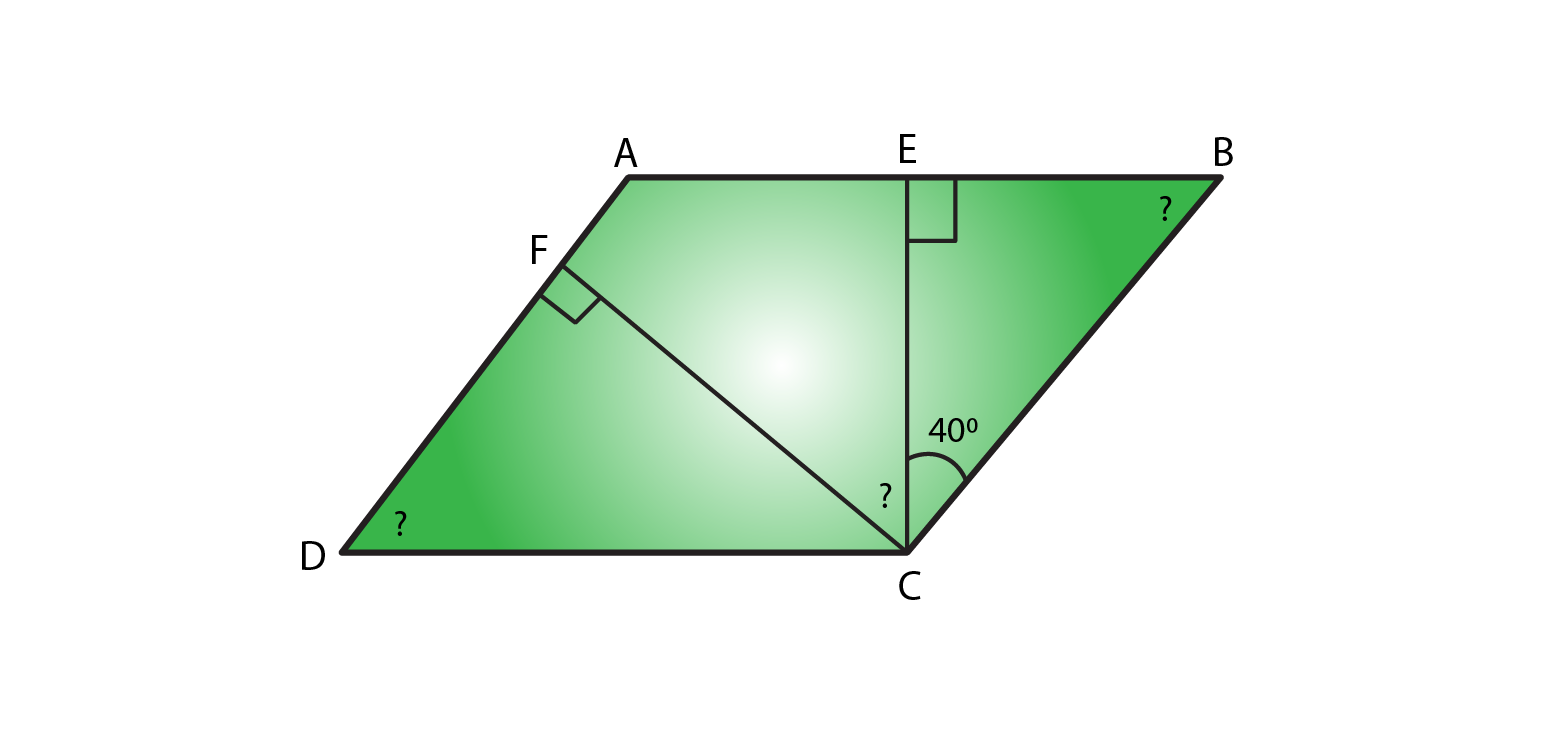Solution:

In ΔBEC

∠BEC + ∠ECB +∠CBE = 180° [Sum of angles of a triangle is 180°]

90° + 40° + ∠CBE = 180°

∠CBE = 180°-130°

∠CBE = 50°

∠CBE = ∠ADC = 50° (Opposite angles of a parallelogram are equal)

∠B = ∠D = 50° [Opposite angles of a parallelogram are equal]

∠A + ∠B = 180° [Sum of adjacent angles of a triangle is 180°]

∠A + 50° = 180°

∠A = 180°-50°

So, ∠A = 130°

In ΔDFC

∠DFC + ∠FCD +∠CDF = 180° [Sum of angles of a triangle is 180°]

90° + ∠FCD + 50° = 180°

∠FCD = 180°-140°

∠FCD = 40°

∠A = ∠C = 130° [Opposite angles of a parallelogram are equal]

∠C = ∠FCE +∠BCE + ∠FCD

∠FCD + 40° + 40° = 130°

∠FCD = 130° – 80°

∠FCD = 50°

∴ ∠EBC = 50o, ∠ADC = 50o and ∠FCD = 50o

21. The angle between the altitudes of a parallelogram, through the same vertex of an obtuse angle of the parallelogram is 60°. Find the angles of the parallelogram.

Solution: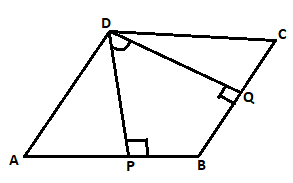Let us consider a parallelogram, ABCD. Where, DP⊥AB and DQ ⊥BC.

Given ∠PDQ = 60°

∠PDQ + ∠DPB + ∠B + ∠BQD = 360° [Sum of all the angles of a Quadrilateral is 360°]

60° + 90° + ∠B + 90° = 360°

∠B = 360° – 240°

∠B = 120°

∠B = ∠D = 120° [Opposite angles of parallelogram are equal]

∠B + ∠C = 180° [Sum of adjacent interior angles in a parallelogram is 180°]

120° + ∠C = 180°

∠C = 180° – 120° = 60°

∠A = ∠C = 60° (Opposite angles of parallelogram are equal)

∴ Angles of a parallelogram are 60o, 120o, 60o, 120o

22. In Figure, ABCD and AEFG are parallelograms. If ∠C =55°, what is the measure of ∠F?Solution:

In parallelogram ABCD

∠C = ∠A = 55° [In a parallelogram opposite angles are equal in a parallelogram]

In parallelogram AEFG

∠A = ∠F = 55° [In a parallelogram opposite angles are equal in a parallelogram]

∴ Measure of ∠F = 55°

23. In Figure, BDEF and DCEF are each a parallelogram. Is it true that BD = DC? Why or why not?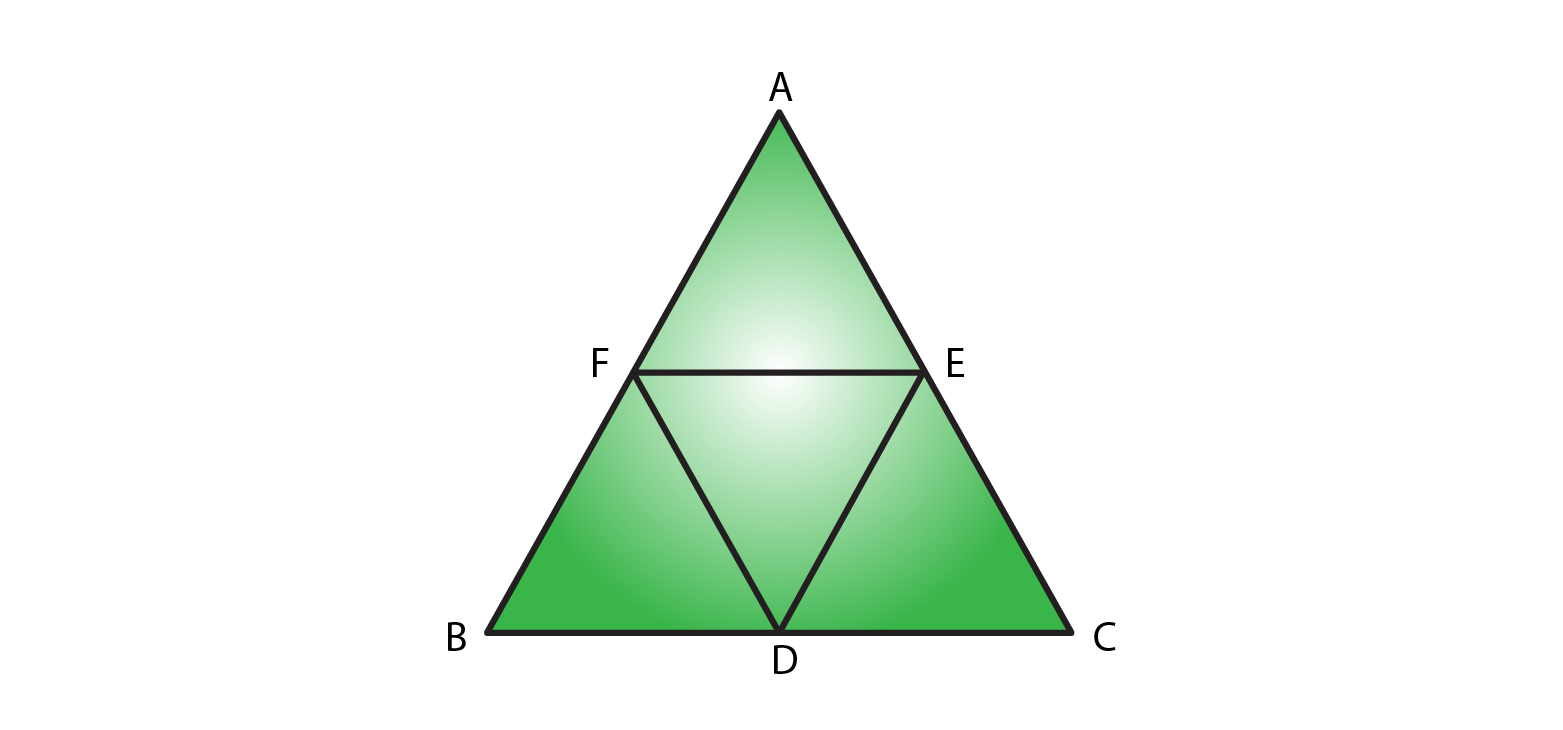Solution:

In parallelogram BDEF

BD = EF [In a parallelogram opposite sides are equal]

In parallelogram DCEF

DC = EF [In a parallelogram opposite sides are equal]

Since, BD = EF = DC

So, BD = DC

24. In Figure, suppose it is known that DE = DF. Then, is ΔABC isosceles? Why or why not?Solution:

In parallelogram BDEF

BD = EF and BF = DE [opposite sides are equal in a parallelogram]

In parallelogram DCEF

DC = EF and DF = CE [opposite sides are equal in a parallelogram]

In parallelogram AFDE

AF = DE and DF = AE [opposite sides are equal in a parallelogram]

So, DE = AF = BF

Similarly: DF = CE = AE

Given, DE = DF

Since, DF = DF

AF + BF = CE + AE

AB = AC

∴ ΔABC is an isosceles triangle.

25. Diagonals of parallelogram ABCD intersect at O as shown in Figure. XY contains O, and X, Y are points on opposite sides of the parallelogram. Give reasons for each of the following:

(i) OB = OD

(ii) ∠OBY = ∠ODX

(iii) ∠BOY = ∠DOX

(iv) ΔBOY = ΔDOX

Now, state if XY is bisected at O.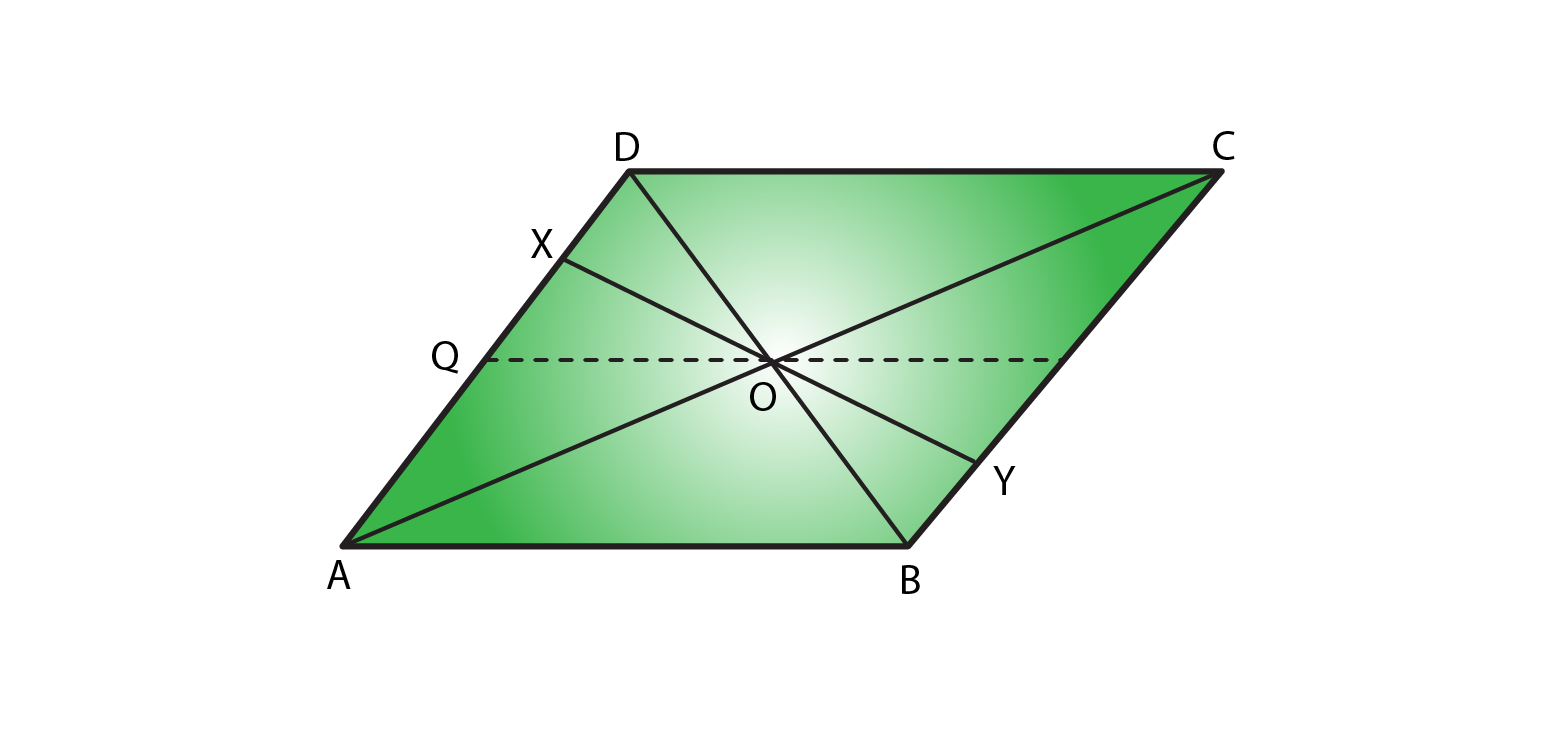Solution:

(i) OB = OD

OB = OD. Since diagonals bisect each other in a parallelogram.

(ii) ∠OBY =∠ODX

∠OBY =∠ODX. Since alternate interior angles are equal in a parallelogram.

(iii) ∠BOY= ∠DOX

∠BOY= ∠DOX. Since vertical opposite angles are equal in a parallelogram.

(iv) ΔBOY ≅ ΔDOX

ΔBOY and ΔDOX. Since OB = OD, where diagonals bisect each other in a parallelogram.

∠OBY =∠ODX [Alternate interior angles are equal]

∠BOY= ∠DOX [Vertically opposite angles are equal]

ΔBOY ≅ΔDOX [by ASA congruence rule]

OX = OY [Corresponding parts of congruent triangles]

∴ XY is bisected at O.

26. In Fig. 17.31, ABCD is a parallelogram, CE bisects ∠C and AF bisects ∠A. In each of the following, if the statement is true, give a reason for the same:

(i) ∠A = ∠C

(ii) ∠FAB = ½ ∠A

(iii) ∠DCE = ½ ∠C

(iv) ∠CEB = ∠FAB

(v) CE ∥ AF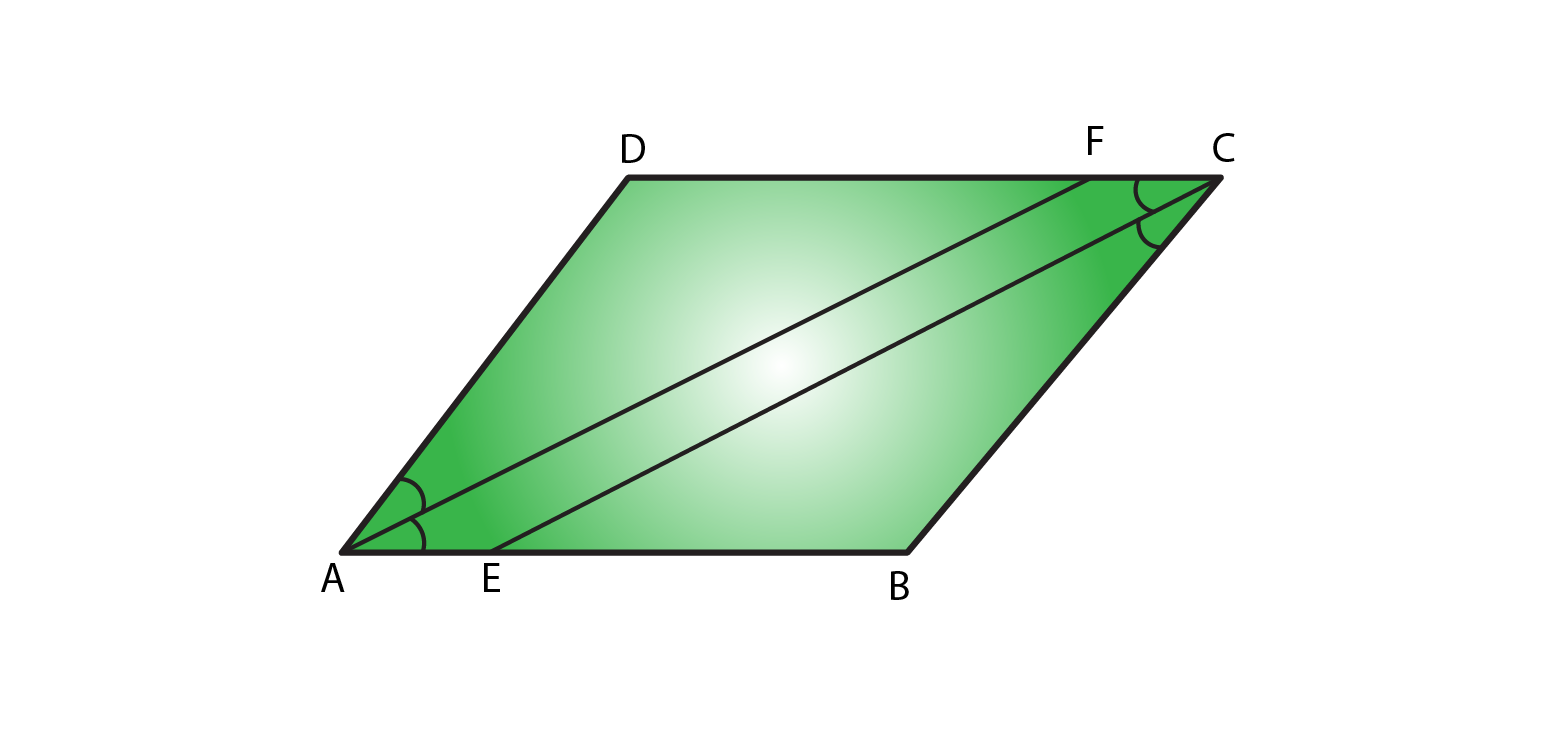Solution:

(i) ∠A = ∠C

True, Since ∠A =∠C = 55° [opposite angles are equal in a parallelogram]

(ii) ∠FAB = ½ ∠A

True, Since AF is the angle bisector of ∠A.

(iii) ∠DCE= ½ ∠C

True, Since CE is the angle bisector of angle ∠C.

(iv) ∠CEB= ∠FAB

True,

Since ∠DCE = ∠FAB (opposite angles are equal in a parallelogram).

∠CEB = ∠DCE (alternate angles)

½ ∠C = ½ ∠A [AF and CE are angle bisectors]

(v) CE || AF

True, since one pair of opposite angles are equal, therefore quad. AEFC is a parallelogram.

27. Diagonals of a parallelogram ABCD intersect at O. AL and CM are drawn perpendiculars to BD such that L and M lie on BD. Is AL = CM? Why or why not?

Solution: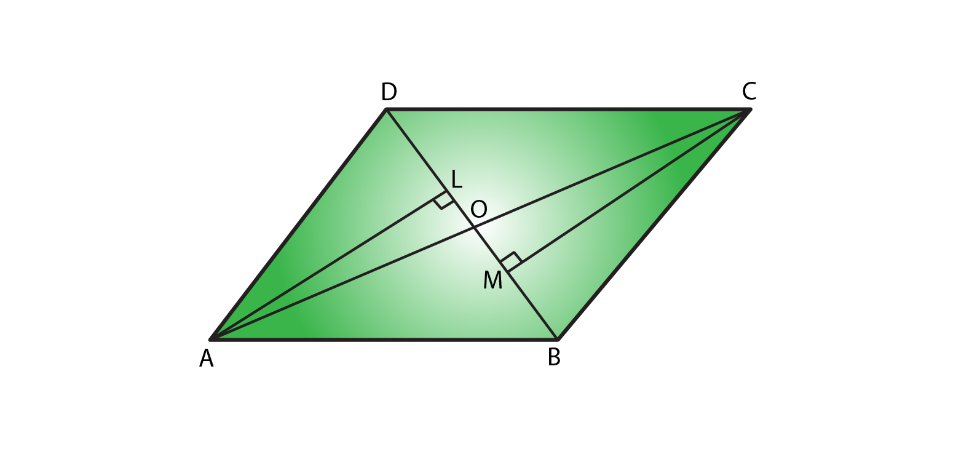Given, AL and CM are perpendiculars on diagonal BD.

In ΔAOL and ΔCOM

∠AOL = ∠COM (vertically opposite angle) ….. (i)

∠ALO = ∠CMO = 90o (each right angle) ……. (ii)

By using angle sum property

∠AOL + ∠ALO + ∠LAO = 180o ……… (iii)

∠COM + ∠CMO + ∠OCM = 180o ……. (iv)

From (iii) and (iv)

∠AOL + ∠ALO + ∠LAO = ∠COM + ∠CMO + ∠OCM

∠LAO = ∠OCM (from (i) and (ii))

In ΔAOL and ΔCOM

∠ALO = ∠CMO (each right angle)

AO = OC (diagonals of a parallelogram bisect each other)

∠LAO = ∠OCM (proved)

So, ΔAOL is congruent to ΔCOM

∴ AL = CM (Corresponding parts of congruent triangles)

28. Points E and F lie on diagonals AC of a parallelogram ABCD such that AE = CF. what type of quadrilateral is BFDE?

Solution: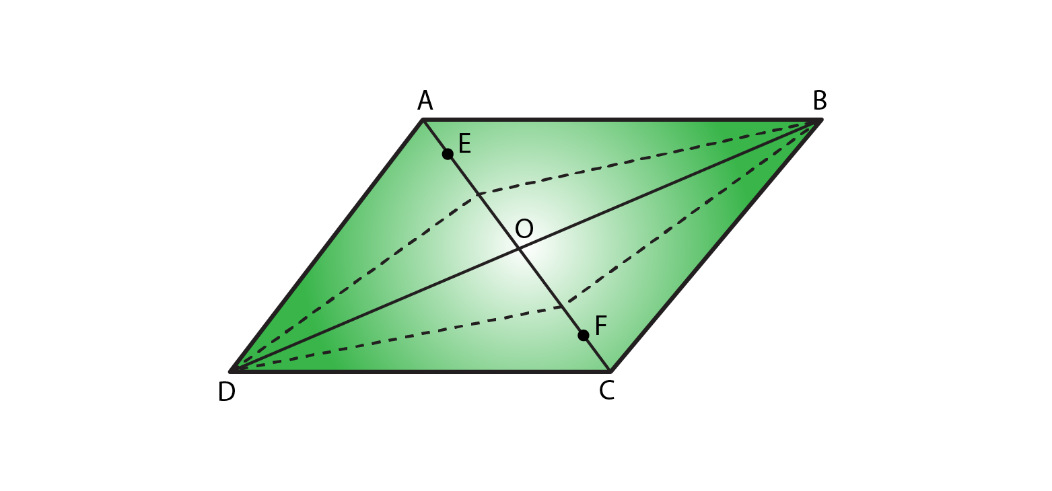In parallelogram ABCD:

AO = OC…………….. (i) (Diagonals of a parallelogram bisect each other)

AE = CF…….. (ii) Given

On subtracting (ii) from (i)

AO – AE = OC – CF

EO = OF ….. (iii)

In ΔDOE and ΔBOF

EO = OF (proved)

DO = OB (Diagonals of a parallelogram bisect each other)

∠DOE = ∠BOF (vertically opposite angles are equal in a parallelogram)

By the rule of SAS congruence ΔDOE ≅ ΔBOF

So, DE = BF (Corresponding parts of congruent triangles)

In ΔBOE and ΔDOF

EO = OF (proved)

DO = OB (diagonals of a parallelogram bisect each other)

∠DOF = ∠BOE (vertically opposite angles are equal in a parallelogram)

By the rule of SAS congruence ΔDOE ≅ ΔBOF

∴ DF = BE (Corresponding parts of congruent triangles)

∴ BFDE is a parallelogram, since one pair of opposite sides are equal and parallel.

29. In a parallelogram ABCD, AB = 10cm, AD = 6 cm. The bisector of ∠A meets DC in E, AE and BC produced meet at F. Find the length CF.

Solution: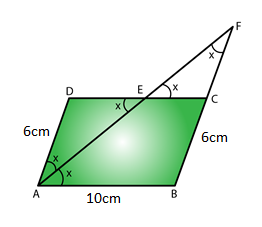In a parallelogram ABCD

Given, AB = 10 cm, AD = 6 cm

⇒ CD = AB = 10 cm and AD = BC = 6 cm [In a parallelogram opposite sides are equal]

AE is the bisector of ∠DAE = ∠BAE = x

∠BAE = ∠AED = x (alternate angles are equal)

ΔADE is an isosceles triangle. Since opposite angles in ΔADE are equal.

AD = DE = 6cm (opposite sides are equal)

CD = DE + EC

EC = CD – DE

= 10 – 6

= 4cm

∠DEA = ∠CEF = x (vertically opposite angle are equal)

∠EAD = ∠EFC = x (alternate angles are equal)

ΔEFC is an isosceles triangle. Since opposite angles in ΔEFC are equal.

CF = CE = 4cm (opposite side are equal to angles)

∴ CF = 4cm.

EXERCISE 17.2 PAGES NO: 17.16

1. Which of the following stat
ements are true for a rhombus?
(i) It has two pairs of parallel sides.
(ii) It has two pairs of equal sides.
(iii) It has only two pairs of equal sides.
(iv) Two of its angles are at right angles.
(v) Its diagonals bisect each other at right angles.
(vi) Its diagonals are equal and perpendicular.
(vii) It has all its sides of equal lengths.
(viii) It is a parallelogram.
(ix) It is a quadrilateral.
(x) It can be a square.
(xi) It is a square.

Solution:

(i) It has two pairs of parallel sides.

True, Rhombus is a parallelogram having two pairs of parallel sides.

(ii) It has two pairs of equal sides.

True, Rhombus has two pairs of equal sides .

(iii) It has only two pairs of equal sides.

False, Rhombus has all four sides equal.

(iv) Two of its angles are at right angles.

False, rhombus has no angle of right angle.

(v) Its diagonals bisect each other at right angles.

True, in rhombus diagonals bisect each other at right angles.

(vi) Its diagonals are equal and perpendicular.

False, in rhombus diagonals are of unequal length.

(vii) It has all its sides of equal lengths.

True, Rhombus has all four sides equal.

(viii) It is a parallelogram.

True, Rhombus is a parallelogram since opposite sides equal and parallel.

(ix) It is a quadrilateral.

True, Rhombus is a quadrilateral since it has four sides.

(x) It can be a square.

True, Rhombus becomes square when any one angle is 90°.

(xi) It is a square.

False, Rhombus is never a square. Since in a square each angle is 90°.

2. Fill in the blanks, in each of the following, so as to make the statement true:
(i) A rhombus is a parallelogram in which _______.
(ii) A square is a rhombus in which _________.
(iii) A rhombus has all its sides of ______ length.
(iv) The diagonals of a rhombus _____ each other at ______ angles.
(v) If the diagonals of a parallelogram bisect each other at right angles, then it is a ______.

Solution:

(i) A rhombus is a parallelogram in which adjacent sides are equal.

(ii) A square is a rhombus in which one angle is right angle.

(iii) A rhombus has all its sides of equal length.

(iv) The diagonals of a rhombus bisect each other at right angles.

(v) If the diagonals of a parallelogram bisect each other at right angles, then it is a rhombus.

3. The diagonals of a parallelogram are not perpendicular. Is it a rhombus? Why or why not?

Solution:

No, Diagonals of a rhombus bisect each other at 90°. Where, diagonals must be perpendicular.

4. The diagonals of a quadrilateral are perpendicular to each other. Is such a quadrilateral always a rhombus? If your answer is ‘No’, draw a figure to justify your answer.

Solution:

No, because diagonals of a rhombus are perpendicular and bisect each other and its sides are equal. Below in the diagram we can see that diagonals are perpendicular to each other but they don’t bisect each other.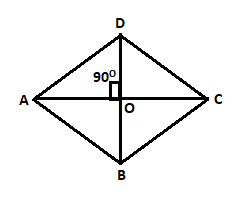5. ABCD is a rhombus. If ∠ACB = 40°, find ∠ADB.

Solution: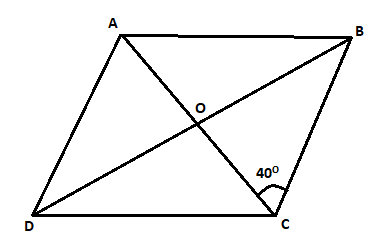ABCD is a rhombus. Diagonals are perpendicular.

So, ∠BOC = 90o

From ΔBOC, the sum of angles is 180o

∠CBO + ∠BOC + ∠OBC = 180o

∠CBO = 180o – ∠BOC – ∠OBC

= 180o – 40o – 90o

= 50o

∴ ∠ADB = ∠CBO = 50o (alternate angles are equal)

6. If the diagonals of a rhombus are 12 cm and 16 cm, find the length of each side.

Solution: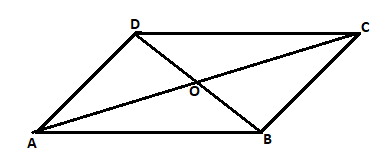We know in rhombus diagonals bisect each other at right angle.

In ΔAOB

AO = 12/2 = 6cm, BO = 16/2 = 8cm

Using Pythagoras theorem in ΔAOB

AB2 = AO2 + BO2

AB2 = 62 + 82

AB2 = 36 + 64

AB2 = 100

AB =√100 = 10cm

∴ Each side of a rhombus is 10cm.

7. Construct a rhombus whose diagonals are of length 10 cm and 6 cm.

Solution: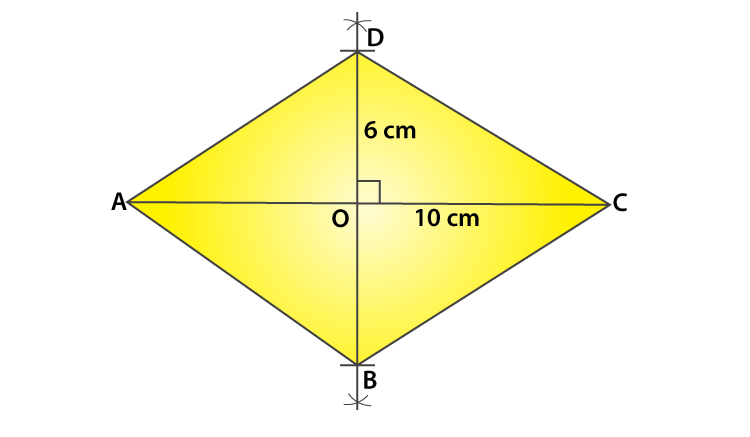Steps to Construct a rhombus:

(i) Draw diagonal AC of length 10 cm.

(ii) With O as centre, draw perpendicular bisector of AC to point O.

(iii) From point ‘O’ cut two arcs of length 3cm each to get points OB and OD.

(iv) Now join AD and AB, BC and CD to get rhombus ABCD.

8. Draw a rhombus, having each side of length 3.5 cm and one of the angles as 40°.

Solution: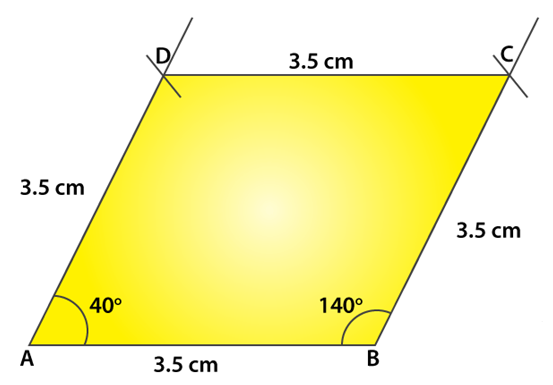Steps to construct a rhombus:

(i) Draw a line segment AB of length 3.5 cm.

(ii) From point A and B draw angles of 40 and 140 respectively.

(iii) From points A and B cut two arcs of length 3.5 cm each to get points D and C.

(iv) Now, join AD, BC, CD to get rhombus ABCD.

9. One side of a rhombus is of length 4 cm and the length of an altitude is 3.2 cm. Draw the rhombus.

Solution: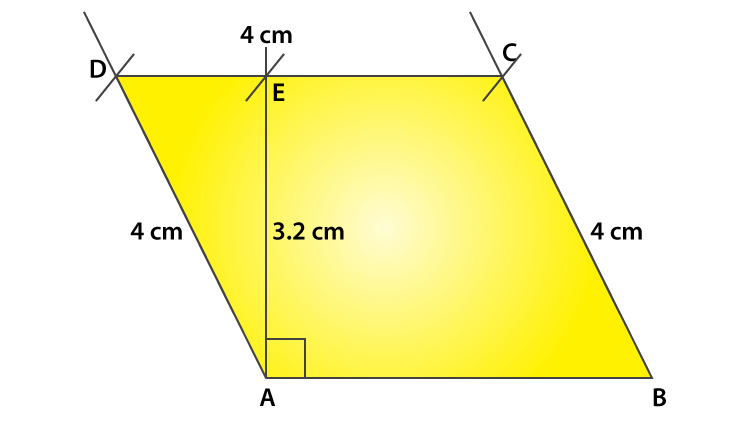Steps to construct a rhombus:

(i) Draw a line segment of 4 cm

(ii) From point A draw a perpendicular line and cut a length of 3.2 cm to get point E.

(iii) From point E draw a line parallel to AB.

(iv) From points A and B cut two arcs of length 4 cm on the drawn parallel line to get points D and C.

(v) Join AD, BC and CD to get rhombus ABCD.

10. Draw a rhombus ABCD, if AB = 6 cm and AC = 5 cm.

Solution: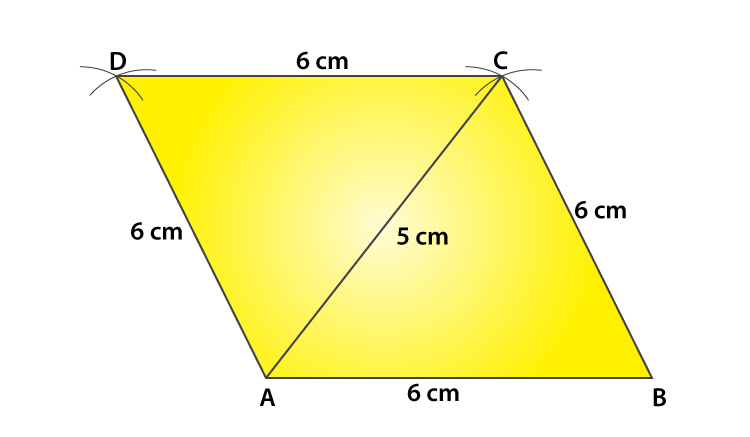Steps of construction:

(i) Draw a line segment AB of length 6 cm.

(ii) From point ‘A’ cut an arc of length 5 cm and from point B cut an arc of length 6 cm. Such that both the arcs intersect at ‘C’.

(iii) Join AC and BC.

(iv) From point A cut an arc of length 6 cm and from point C cut an arc of 6cm, so that both the arcs intersect at point D.

(v) Join AD and DC to get rhombus ABCD.

11. ABCD is a rhombus and its diagonals intersect at O.
(i) is ΔBOC ≅ ΔDOC? State the congruence condition used?
(ii) Also state, if ∠BCO = ∠DCO.

Solution:(i) Yes,

In ΔBOC and ΔDOC

BO = DO [In a rhombus diagonals bisect each other]

CO = CO Common

BC = CD [All sides of a rhombus are equal]

By using SSS Congruency, ΔBOC≅ΔDOC

(ii) Yes,

∠BCO = ∠DCO, by corresponding parts of congruent triangles.

12. Show that each diagonal of a rhombus bisects the angle through which it passes.

Solution:

(i) In ΔBOC and ΔDOC

BO = DO [In a rhombus diagonals bisect each other]

CO = CO Common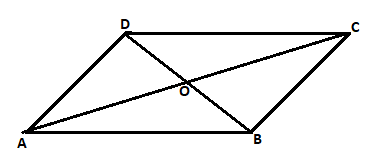BC = CD [All sides of a rhombus are equal]

By using SSS Congruency, ΔBOC≅ΔDOC

∠BCO = ∠DCO, by corresponding parts of congruent triangles

∴ Each diagonal of a rhombus bisect the angle through which it passes.

13. ABCD is a rhombus whose diagonals intersect at O. If AB=10 cm, diagonal BD = 16 cm, find the length of diagonal AC.

Solution:We know in rhombus diagonals bisect each other at right angle.

In ΔAOB

BO = BD/2 = 16/2 = 8cm

Using Pythagoras theorem in ΔAOB

AB2 = AO2 + BO2

102 = AO2 + 82

100-64 = AO2

AO2 = 36

AO = √36 = 6cm

∴ Length of diagonal AC is 6 × 2 = 12cm.

14. The diagonal of a quadrilateral are of lengths 6 cm and 8 cm. If the diagonals bisect each other at right angles, what is the length of each side of the quadrilateral?

Solution: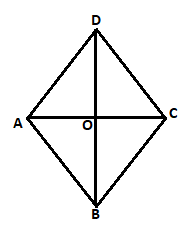We know in rhombus diagonals bisect each other at right angle.

In ΔAOB

BO = BD/2 = 6/2 = 3cm

AO = AC/2 = 8/2 = 4cm

Using Pythagoras theorem in ΔAOB

AB2 = AO2 + BO2

AB2 = 42 + 32

AB2 = 16 + 9

AB2 = 25

AB = √25 = 5cm

∴ Length of each side of a Quadrilateral ABCD is 5cm.

EXERCISE 17.3 PAGES NO: 17.22

1. Which of the following statem
ents are true for a rectangle?
(i) It has two pairs of equal sides.
(ii) It has all its sides of equal length.
(iii) Its diagonals are equal.
(iv) Its diagonals bisect each other.
(v) Its diagonals are perpendicular.
(vi) Its diagonals are perpendicular and bisect each other.
(vii) Its diagonals are equal and bisect each other.
(viii) Its diagonals are equal and perpendicular, and bisect each other.
(ix) All rectangles are squares.
(x) All rhombuses are parallelograms.
(xi) All squares are rhombuses and also rectangles.
(xii) All squares are not parallelograms.

Solution:

(i) It has two pairs of equal sides.

True, in a rectangle two pairs of sides are equal.

(ii) It has all its sides of equal length.

False, in a rectangle only two pairs of sides are equal.

(iii) Its diagonals are equal.

True, in a rectangle diagonals are of equal length.

(iv) Its diagonals bisect each other.

True, in a rectangle diagonals bisect each other.

(v) Its diagonals are perpendicular.

False, Diagonals of a rectangle need not be perpendicular.

(vi) Its diagonals are perpendicular and bisect each other.

False, Diagonals of a rectangle need not be perpendicular. Diagonals only bisect each other.

(vii) Its diagonals are equal and bisect each other.

True, Diagonals are of equal length and bisect each other.

(viii) Its diagonals are equal and perpendicular, and bisect each other.

False, Diagonals are of equal length and bisect each other. Diagonals of a rectangle need not be perpendicular

(ix) All rectangles are squares.

False, in a square all sides are of equal length.

(x) All rhombuses are parallelograms.

True, all rhombuses are parallelograms, since opposite sides are equal and parallel.

(xi) All squares are rhombuses and also rectangles.

True, all squares are rhombuses, since all sides are equal in a square and rhombus. All squares are rectangles, since opposite sides are equal and parallel.

(xii) All squares are not parallelograms.

False, all squares are parallelograms, since opposite sides are parallel and equal.

2. Which of the following sta
tements are true for a square?
(i) It is a rectangle.
(ii) It has all its sides of equal length.
(iii) Its diagonals bisect each other at right angle.
(v) Its diagonals are equal to its sides.

Solution:

(i) It is a rectangle.

True. Since, opposite sides are equal and parallel where, each angle is right angle.

(ii) It has all its sides of equal length.

True. Since, ides of a square are of equal length.

(iii) Its diagonals bisect each other at right angle.

True. Since, diagonals of a square bisect each other at right angle.

(v) Its diagonals are equal to its sides.

False. Since, diagonals of a square are of equal length. Length of diagonals is not equal to the length of sides

3. Fill in the blanks in each of the following, so as to make the statement true :
(i) A rectangle is a parallelogram in which ________.
(ii) A square is a rhombus in which __________.
(iii) A square is a rectangle in which ___________.

Solution:

(i) A rectangle is a parallelogram in which one angle is a right angle.

(ii) A square is a rhombus in which one angle is a right angle.

(iii) A square is a rectangle in which adjacent sides are equal.

4. A window frame has one diagonal longer than the other. Is the window frame a rectangle? Why or why not?

Solution:

No, diagonals of a rectangle are equal length.

5. In a rectangle ABCD, prove that ΔACB ≅ΔCAD.

Solution:

Let us draw a rectangle,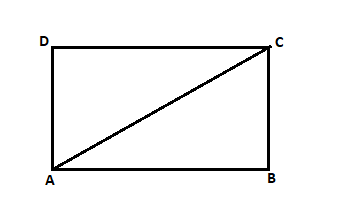In rectangle ABCD, AC is the diagonal.

In ΔACB and ΔCAD

AB = CD [Opposite sides of a rectangle are equal]

BC = DA

AC = CA [Common]

By using SSS congruency

6. The sides of a rectangle are in the ratio 2 : 3, and its perimeter is 20 cm. Draw the rectangle.

Solution:

In rectangle ABCD,

Given, perimeter of a rectangle = 20cm

Ratio = 2:3

So, let us consider the side as ‘x’

Length of rectangle (l) = 3x

Breadth of the rectangle (b) = 2x

We know that,

Perimeter of the rectangle = 2(length + breadth)

20 = 2(3x + 2x)

10x = 20

x = 20/10 = 2

Length of the rectangle = 3×2 = 6cm

Breadth of the rectangle = 2×2 = 4cm

Here, is the diagram of rectangle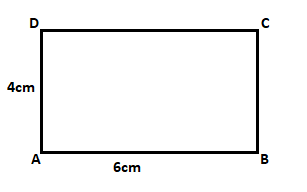7. The sides of a rectangle are in the ratio 4 : 5. Find its sides if the perimeter is 90 cm.

Solution:

In rectangle ABCD,

Given, perimeter of a rectangle = 90cm

Ratio = 4:5

So, let us consider the side as ‘x’

Length of rectangle (l) = 5x

Breadth of the rectangle (b) = 4x

We know that,

Perimeter of the rectangle = 2(length + breadth)

90 = 2(5x + 4x)

18x = 90

x = 90/18 = 5

Length of the rectangle = 5×5 = 25cm

Breadth of the rectangle = 4×5 = 20cm

Here, is the diagram of rectangle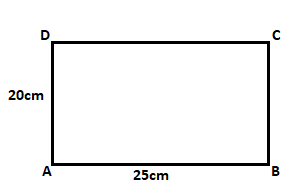8. Find the length of the diagonal of a rectangle whose sides are 12 cm and 5 cm.

Solution: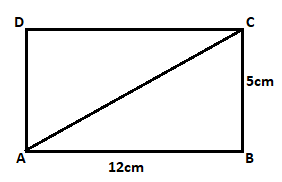In rectangle ABCD,

Given, sides of a rectangle ABCD are 5cm and 12cm

In ΔABC using Pythagoras theorem,

AC2 = AB2 + BC2

AC2 = 122 + 52

AC2 = 144 + 25

AC2 = 169

AC = √169

AC = 13cm

∴ Length of the diagonal AC is 13cm.

9. Draw a rectangle whose one side measures 8 cm and the length of each of whose diagonals is 10 cm.

Solution:

Given, one side of the rectangle is 8cm.

Length of the diagonal = 10cm

Now let us construct a rectangle,

Steps to construct a rectangle,

(i) Draw a line segment AB of length 8 cm

(ii) From point ‘A’ cut an arc of length 10 cm and mark that point as C.

(iii) From point B draw an angle of 90°, and join the arc from point A which cuts at point C.

(iv) now join AC and BC

(v) From point A draw an angle of 90° and from point C cut an arc of length 8 cm to get point D.

(vi) Join CD and AD to form required rectangle.

Here, is the constructed diagram of rectangle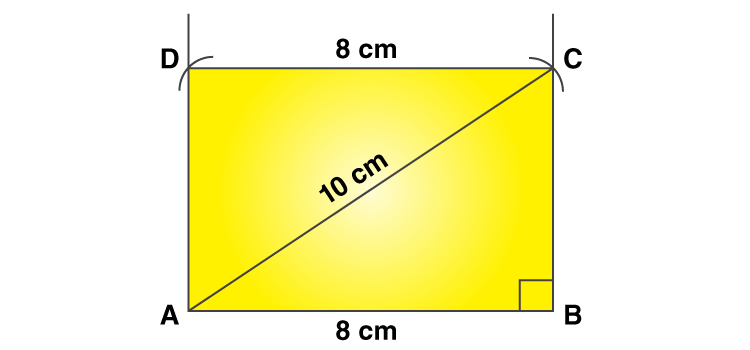10. Draw a square who’s each side measures 4.8 cm.

Solution:

Given, side of a square is 4.8cm.

Now let us construct a square,

Steps to construct a square,

(i) Draw a line segment AB of length 4.8 cm.

(ii) From points A and B draw perpendiculars at 90o each.

(iii) Cut and arc of 4.8 cm from point A and B on the perpendiculars to get point D and C.

(iv) Join DC, AD and BC to form the required square.

Here, is the constructed diagram of square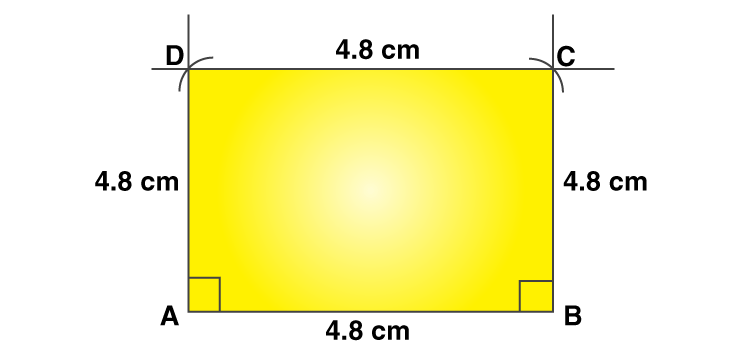11. Identify all the quadrilaterals that have:

(i) Four sides of equal length

(ii) Four right angles

Solution:

(i) Four sides of equal length

The quadrilaterals which has all the four sides of equal length are Square and Rhombus.

(ii) Four right angles

The quadrilaterals which has four right angles are Square and Rectangle.

12. Explain how a square is
(ii) A parallelogram?
(iii) A rhombus?
(iv) A rectangle?

Solution:

(i) A square is a quadrilateral since it has all sides of equal length.

(ii) A square is a parallelogram since it’s opposite sides are equal and parallel.

(iii) A square is a rhombus since it has all sides of equal length and opposite sides are parallel.

(iv) A square is a rectangle since it’s opposite sides are equal and each angle is a 90o.

13. Name the quadrilaterals whose diagonals:
(i) bisect each other
(ii) are perpendicular bisector of each other
(iii) are equal.

Solution:

(i) Quadrilaterals whose diagonals bisect each other are: Parallelogram,

Rectangle, Rhombus and Square.

(ii) Quadrilaterals whose diagonals are perpendicular bisector of each other are: Rhombus and Square.

(iii) Quadrilaterals whose diagonals are equal in Square and Rectangle.

14. ABC is a right angled triangle and O is the mid-point of the side opposite to the right angle. Explain why O is equidistant from A, B, and C.

Solution: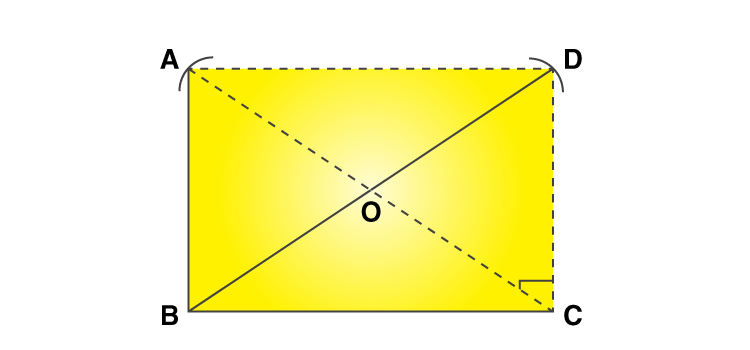ABC is a right angled triangle. O is the midpoint of hypotenuse AC, such that OA = OC

Now, draw CD||AB and join AD, such that AB = CD and AD = BC.

Now, quadrilateral ABCD is a rectangle, since each angle is a right angle and opposite sides are equal and parallel.

We know in a rectangle diagonals are of equal length and they bisect each other.

So, AC = BD

And also, AO = OC = BO = OD

Hence, O is equidistant from A, B and C.

15. A mason has made a concrete slab. He needs it to be rectangular. In what different ways can he make sure that it is rectangular?

Solution:

For a concrete slab to be rectangular the mason has to check,

(i) By measuring each angle and

(ii) By measuring the lengths of diagonals.